# Concise Selina Solutions for Class 9 Maths Chapter 2- Compound Interest [Without Using Formula]

Selina Solutions are useful for students as it helps them in scoring high marks in the examination. The Selina Solutions contain detailed step-by-step explanation of all the problems that come under the Chapter 2, Compound Interest [Without Using Formula], of the Class 9 Selina Textbook.

These solutions are prepared by subject matter experts at BYJU’S, describing the complete method of solving problems. By understanding the concepts used in Selina Solutions for Class 9 Maths, students will be able to clear all their doubts related to “Compound Interest [Without Using Formula]”.

## Download PDF of Selina Solutions for Class 9 Maths Chapter 2:-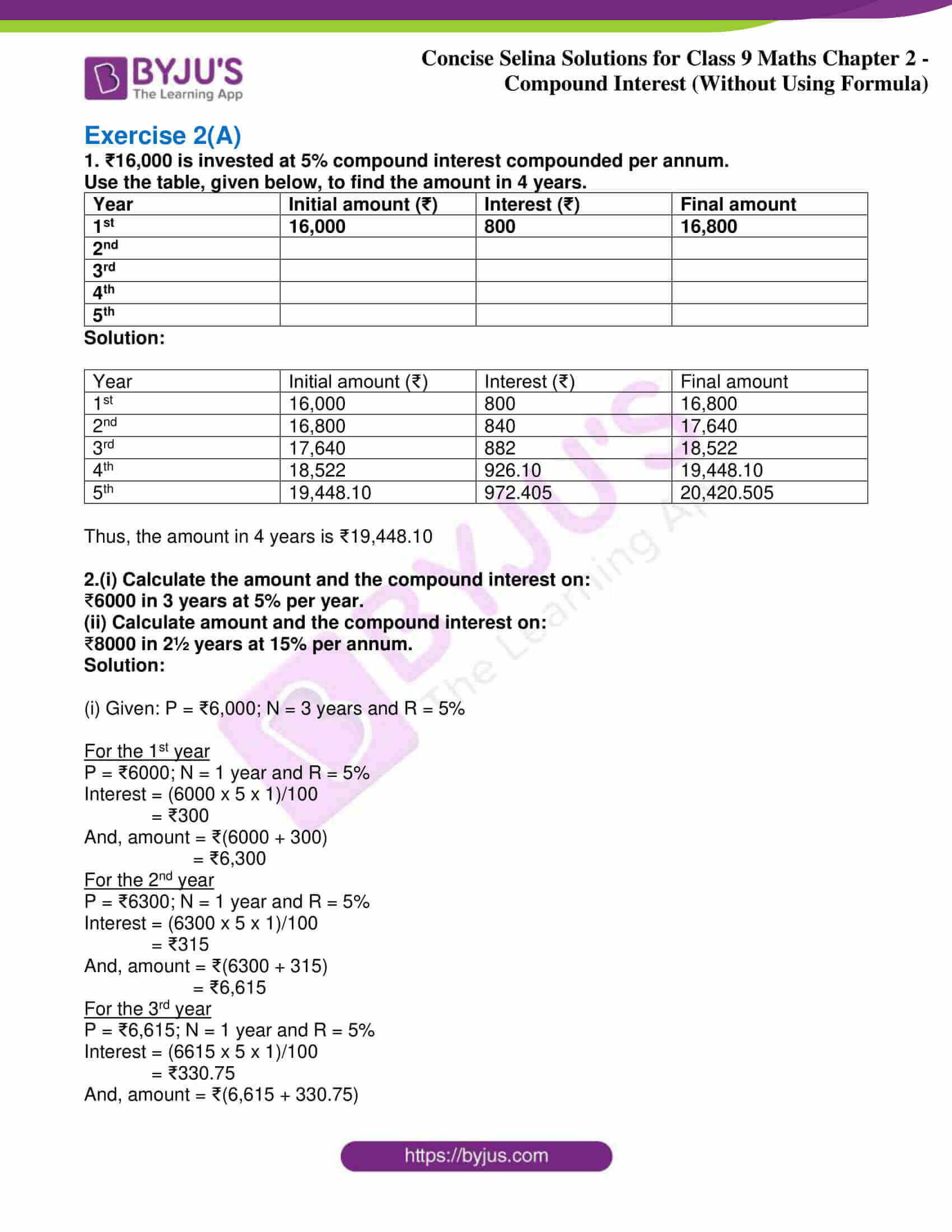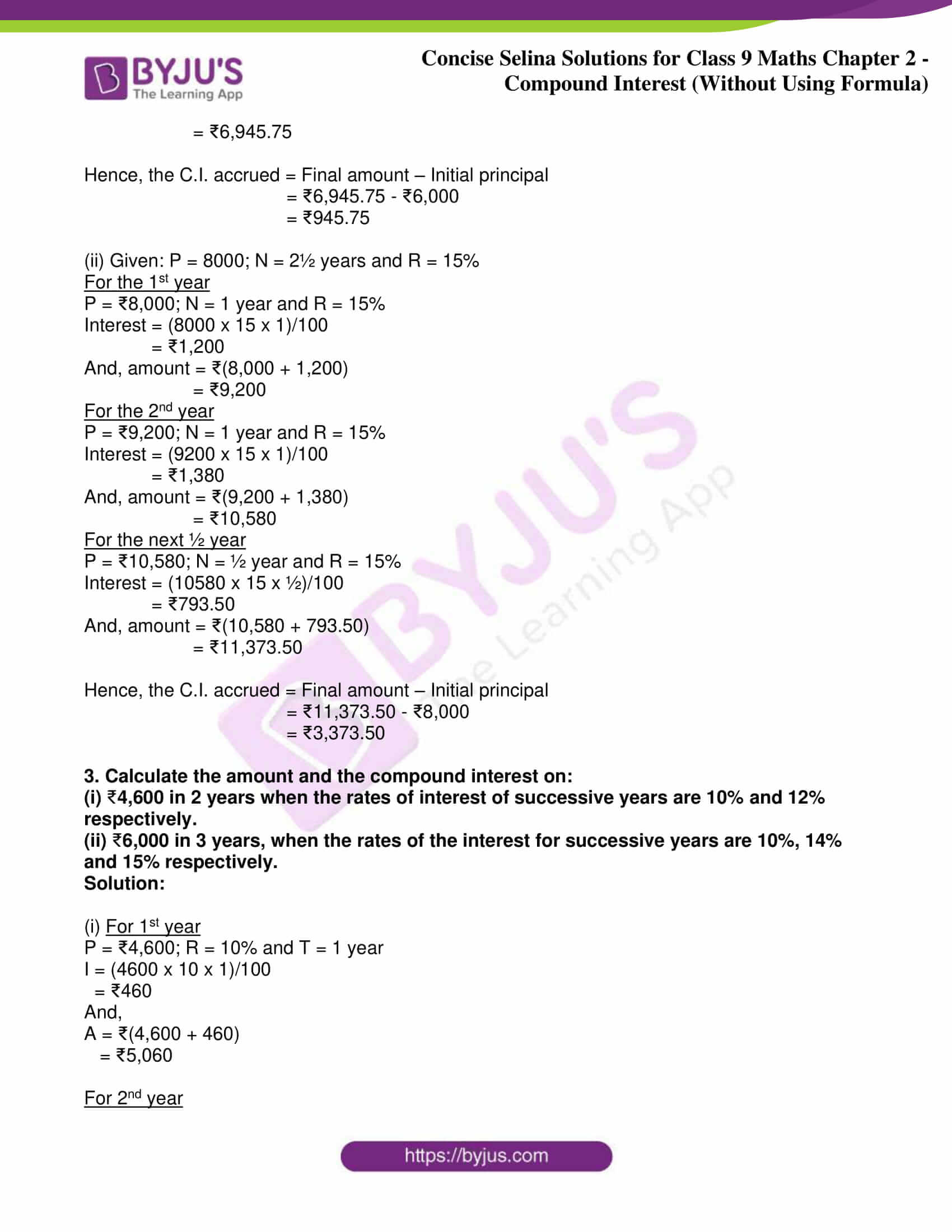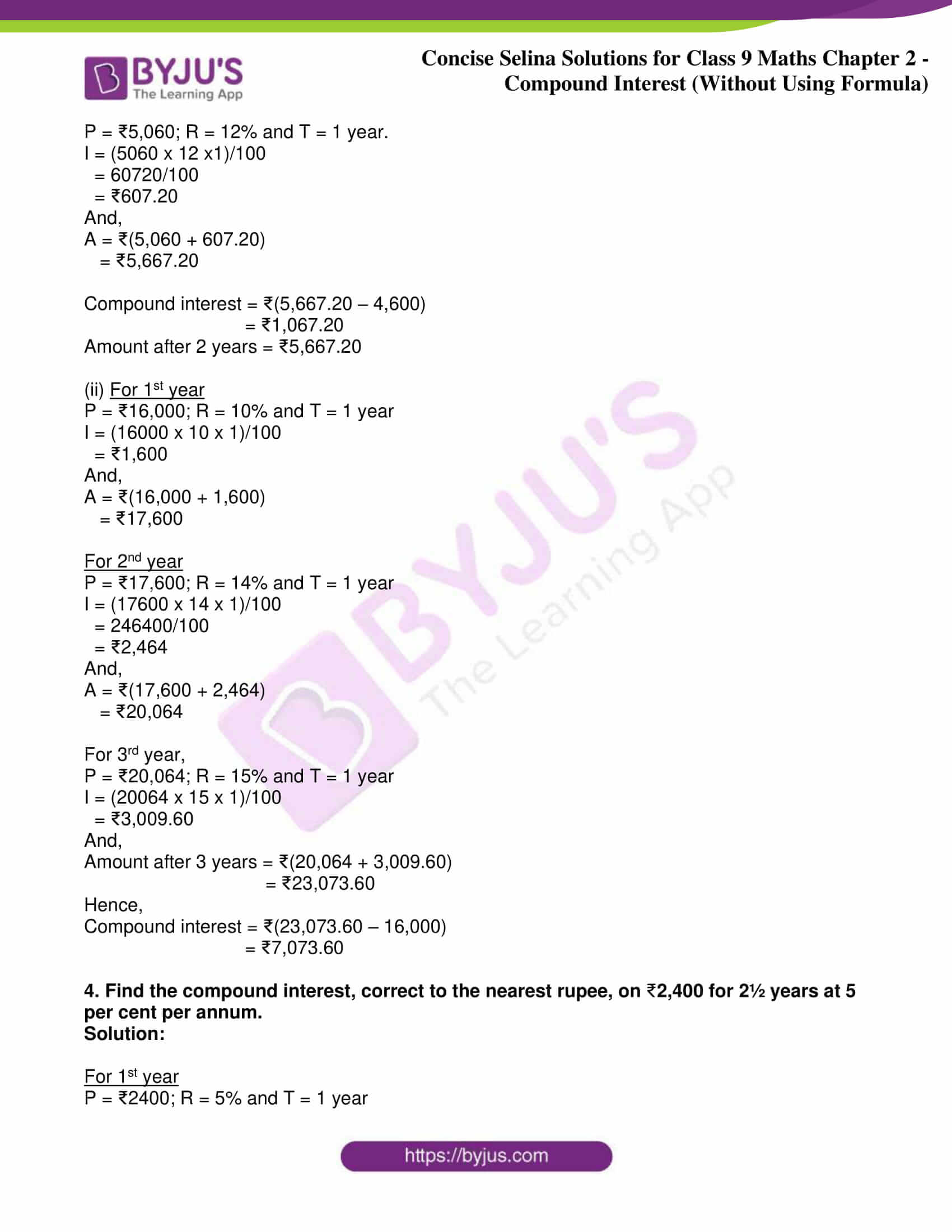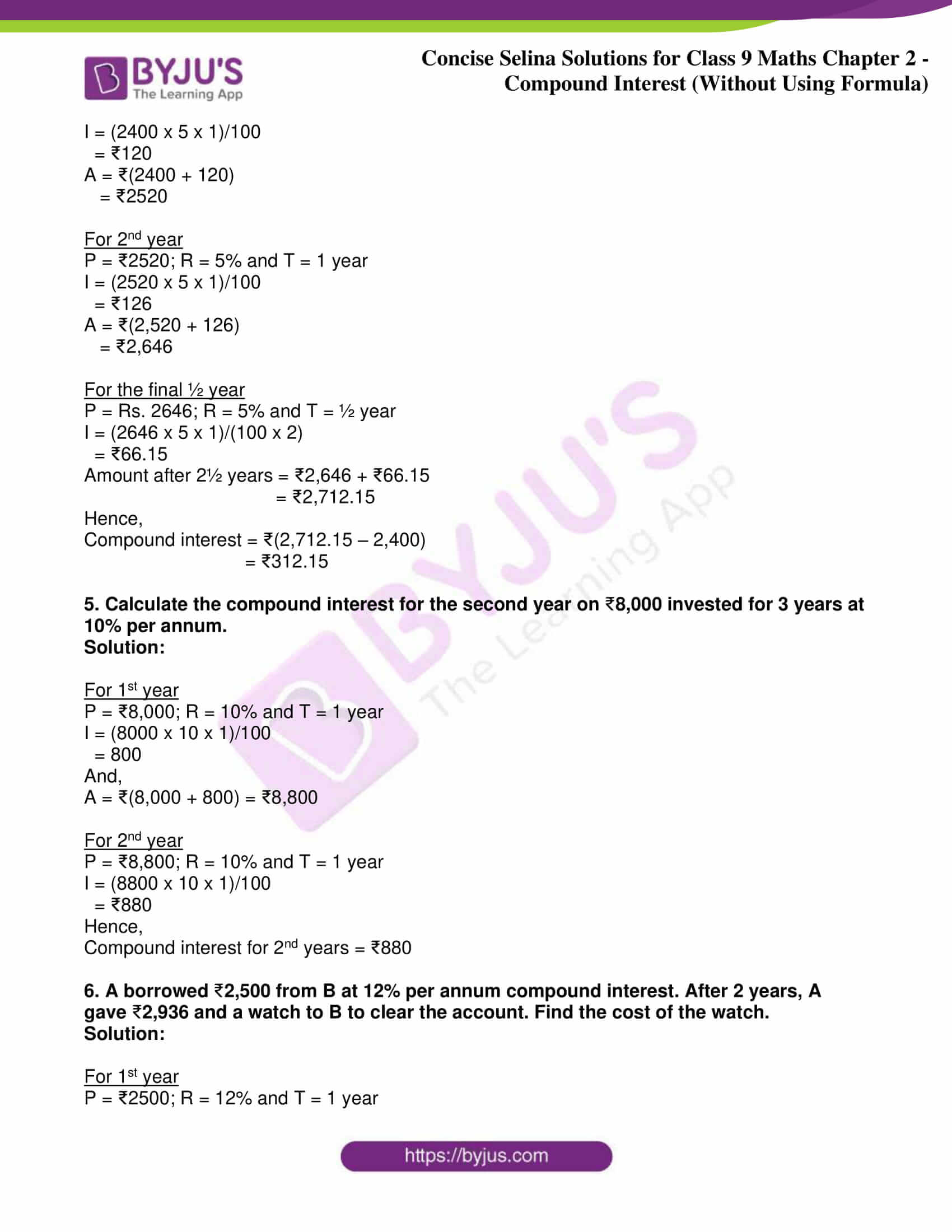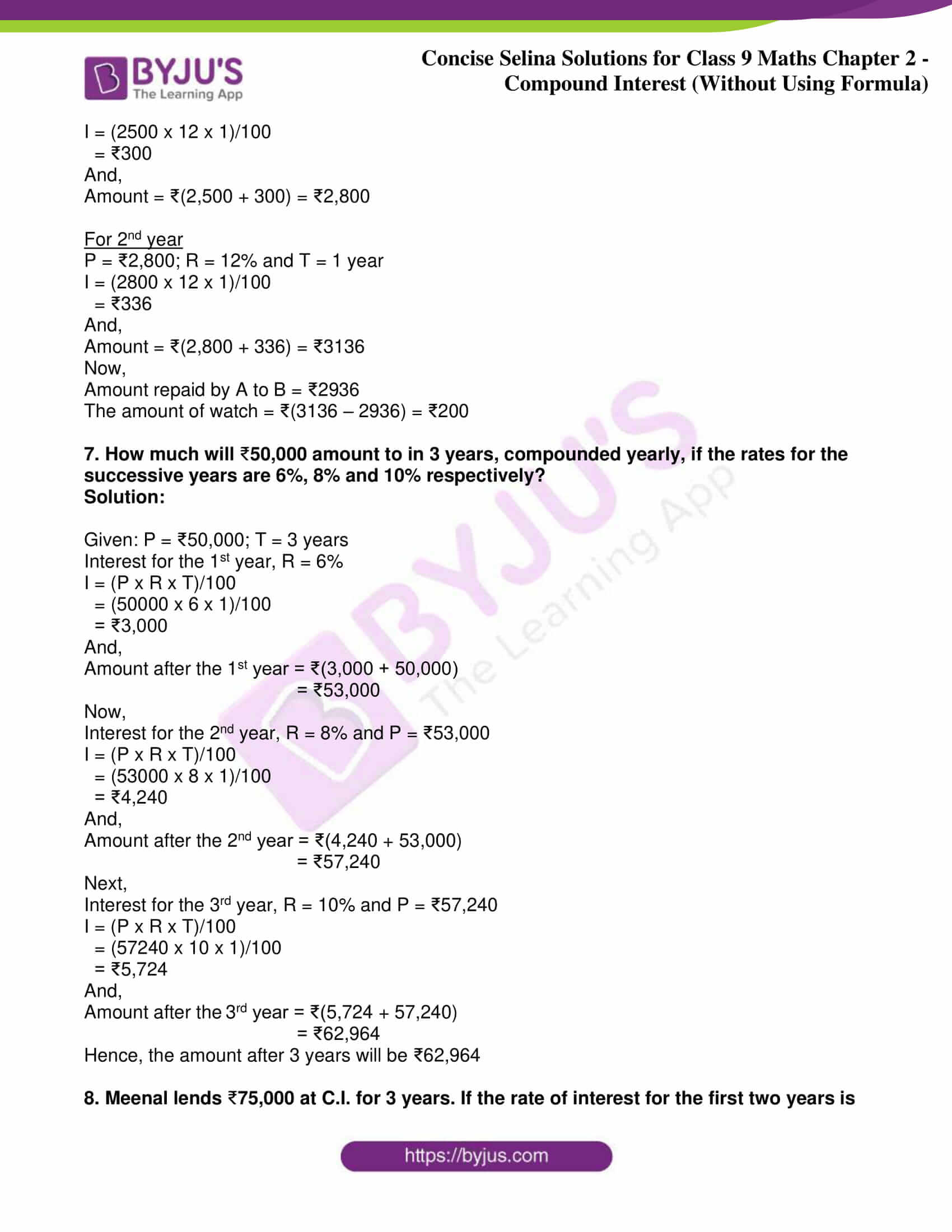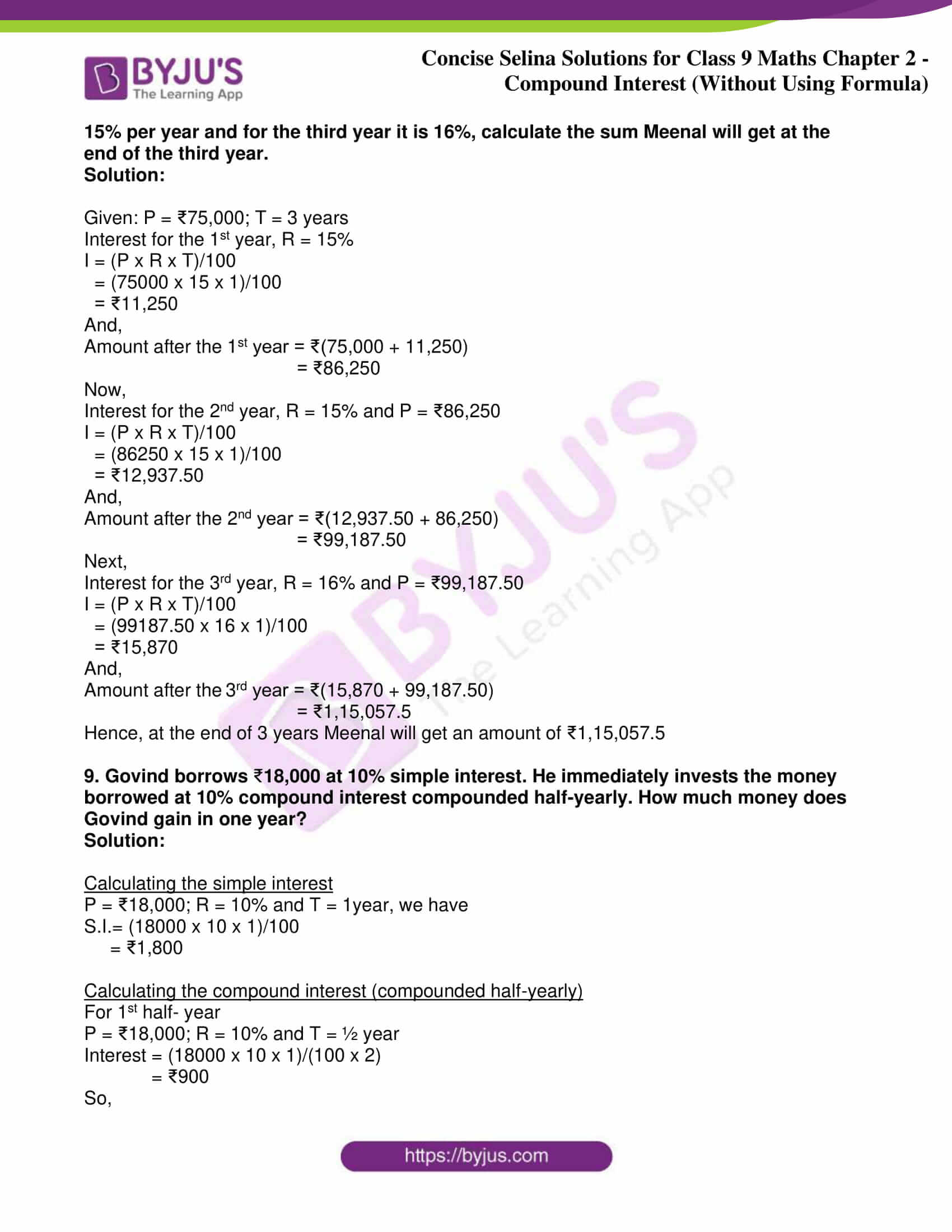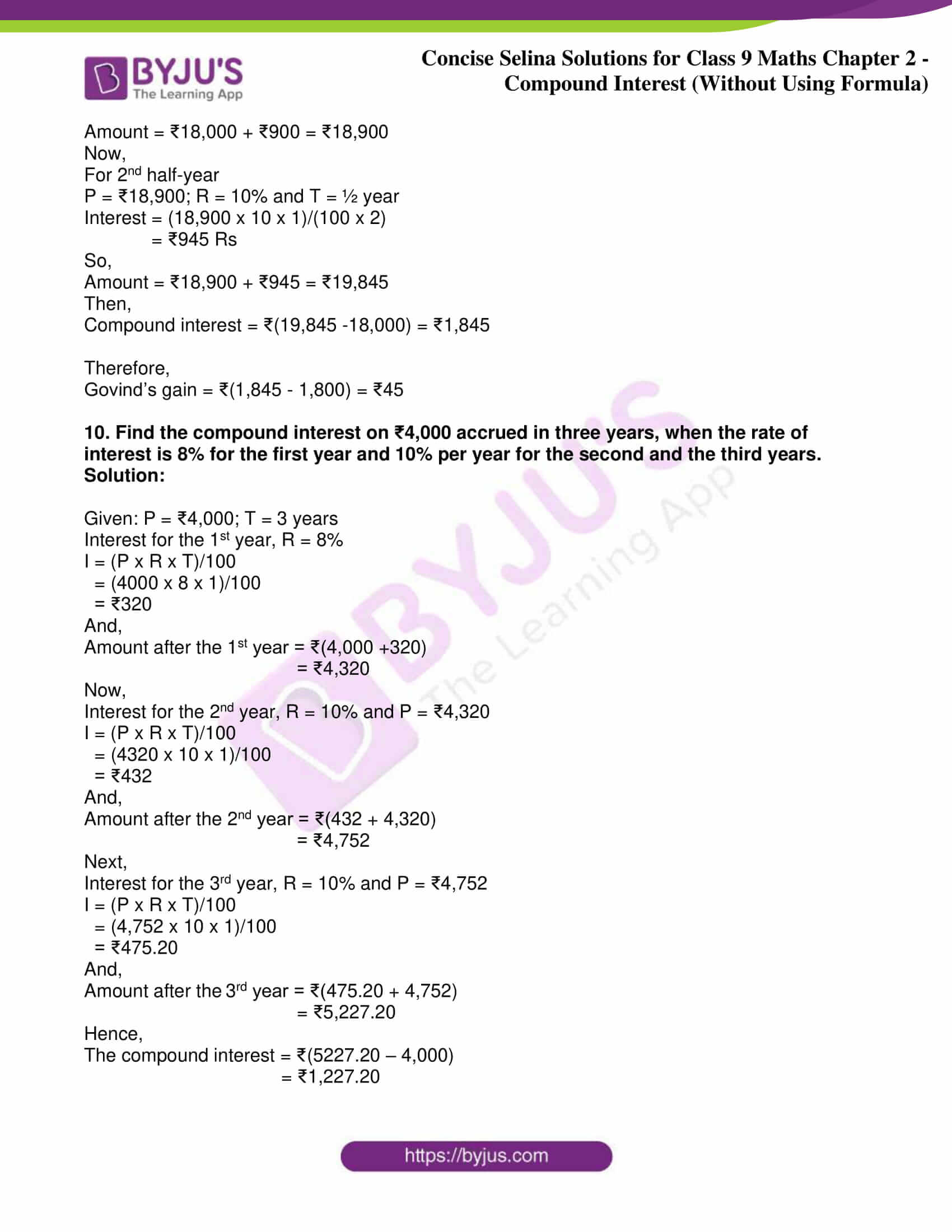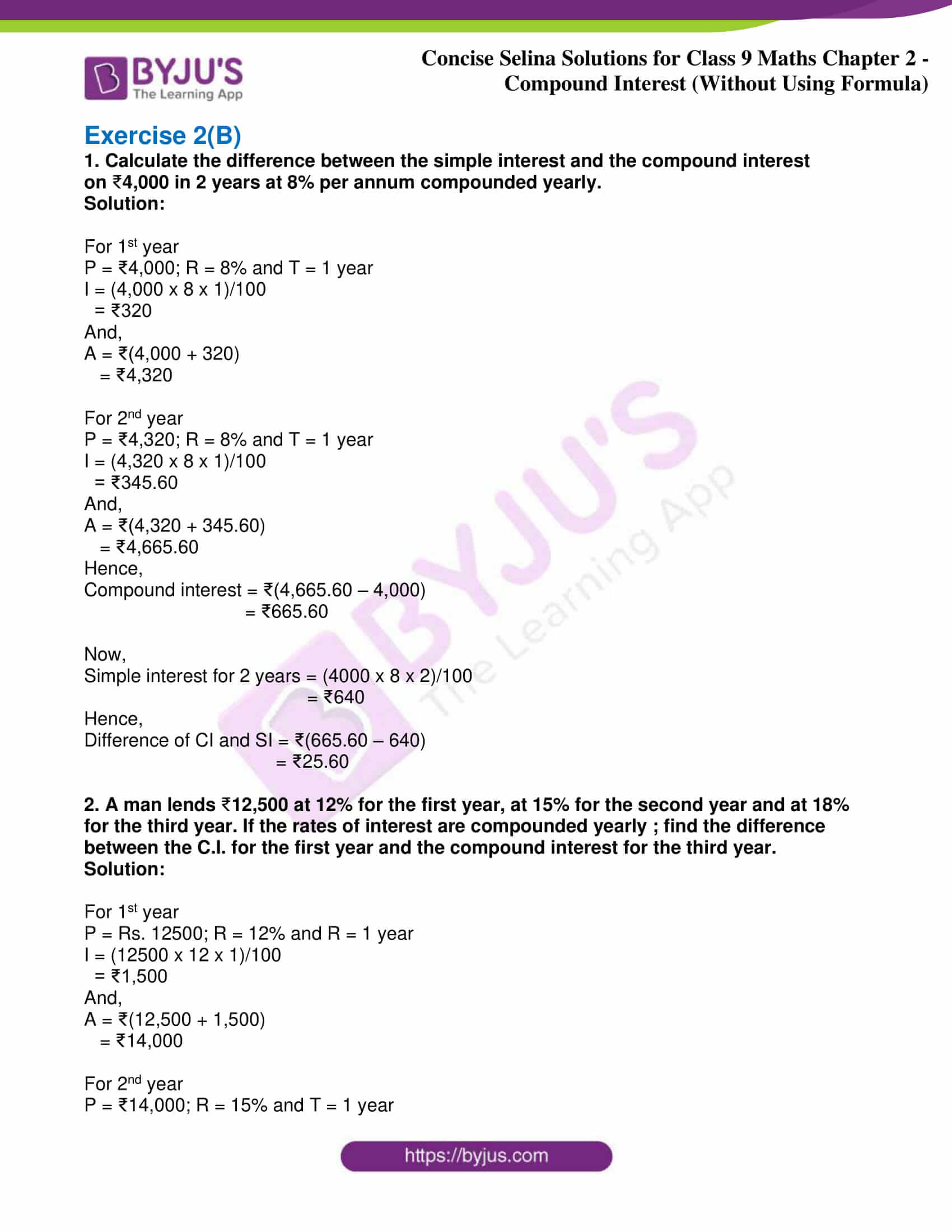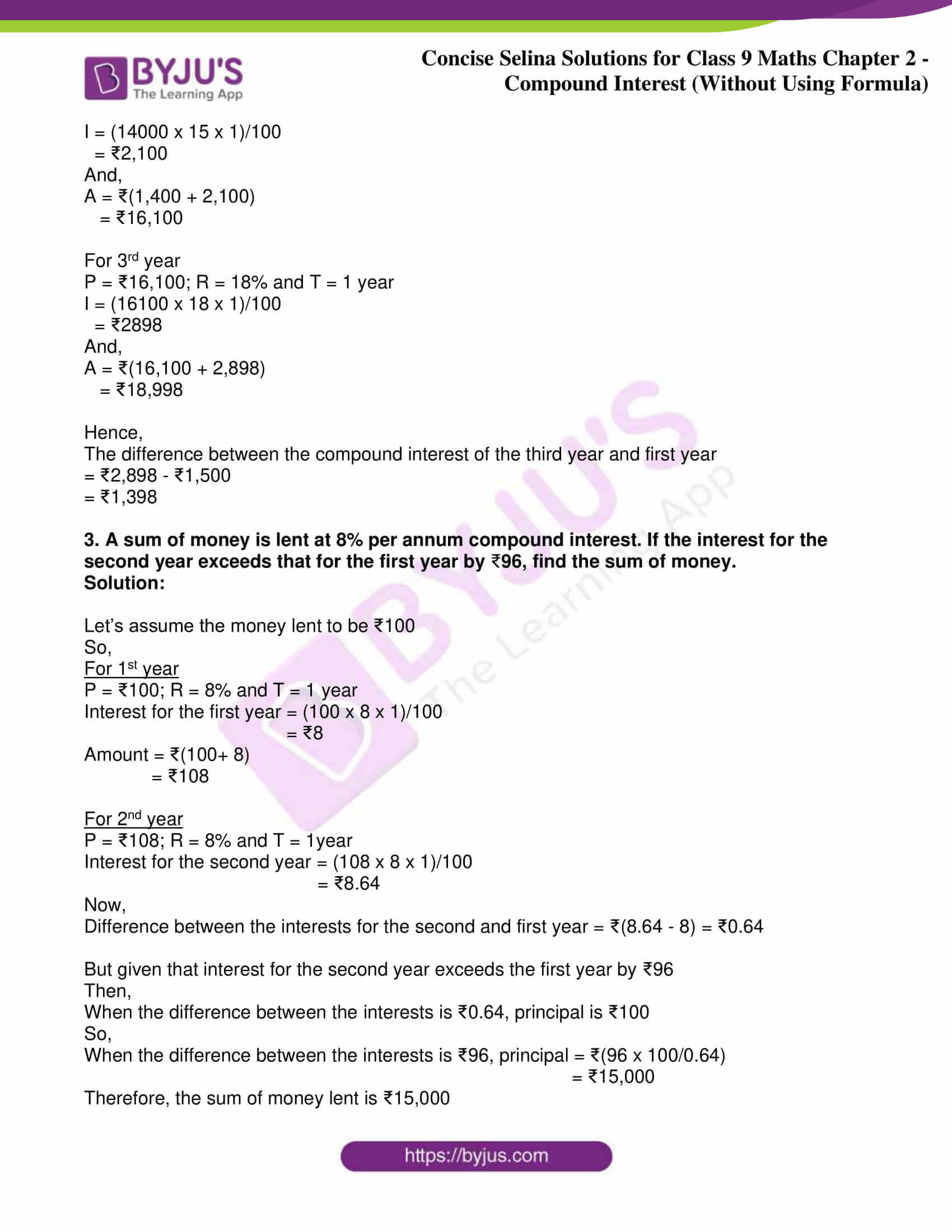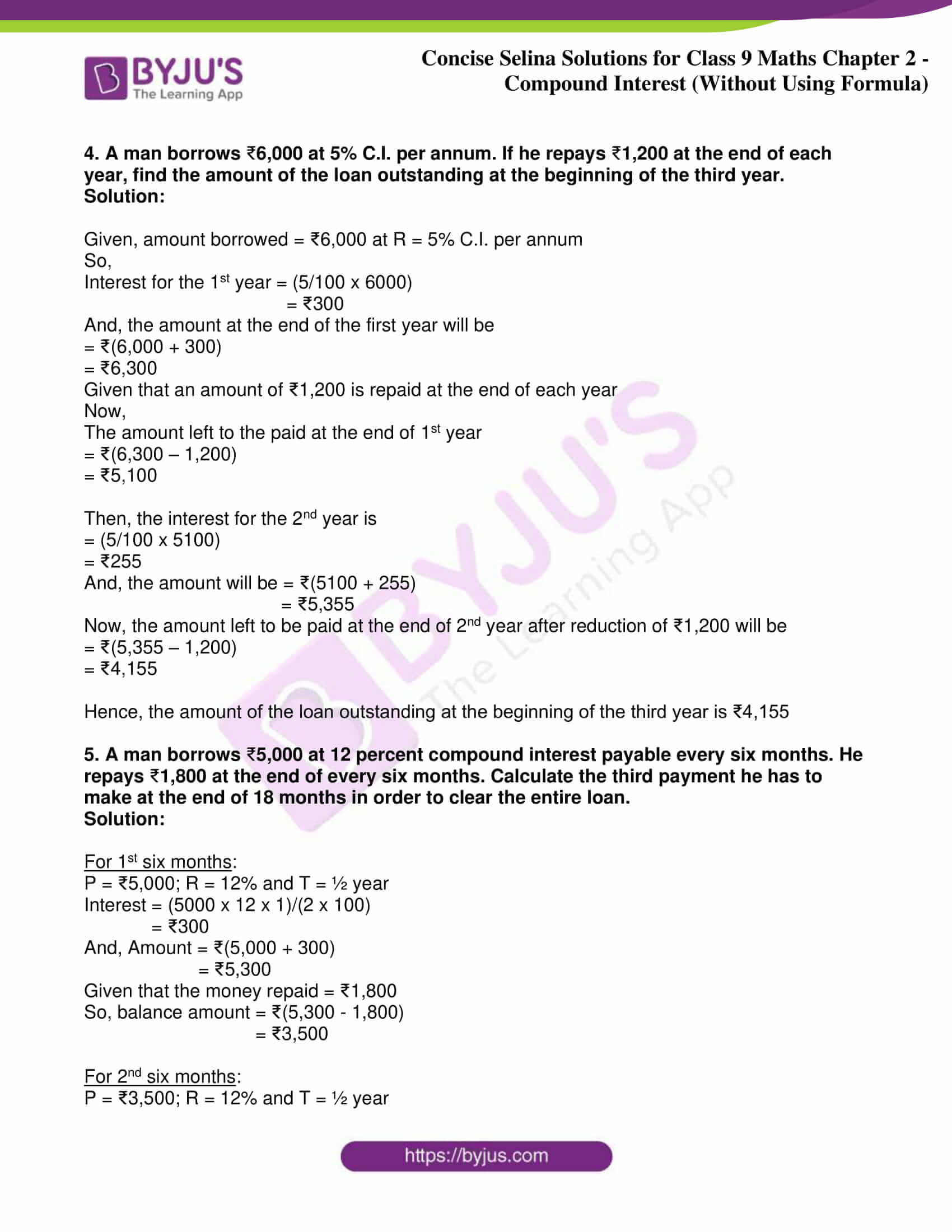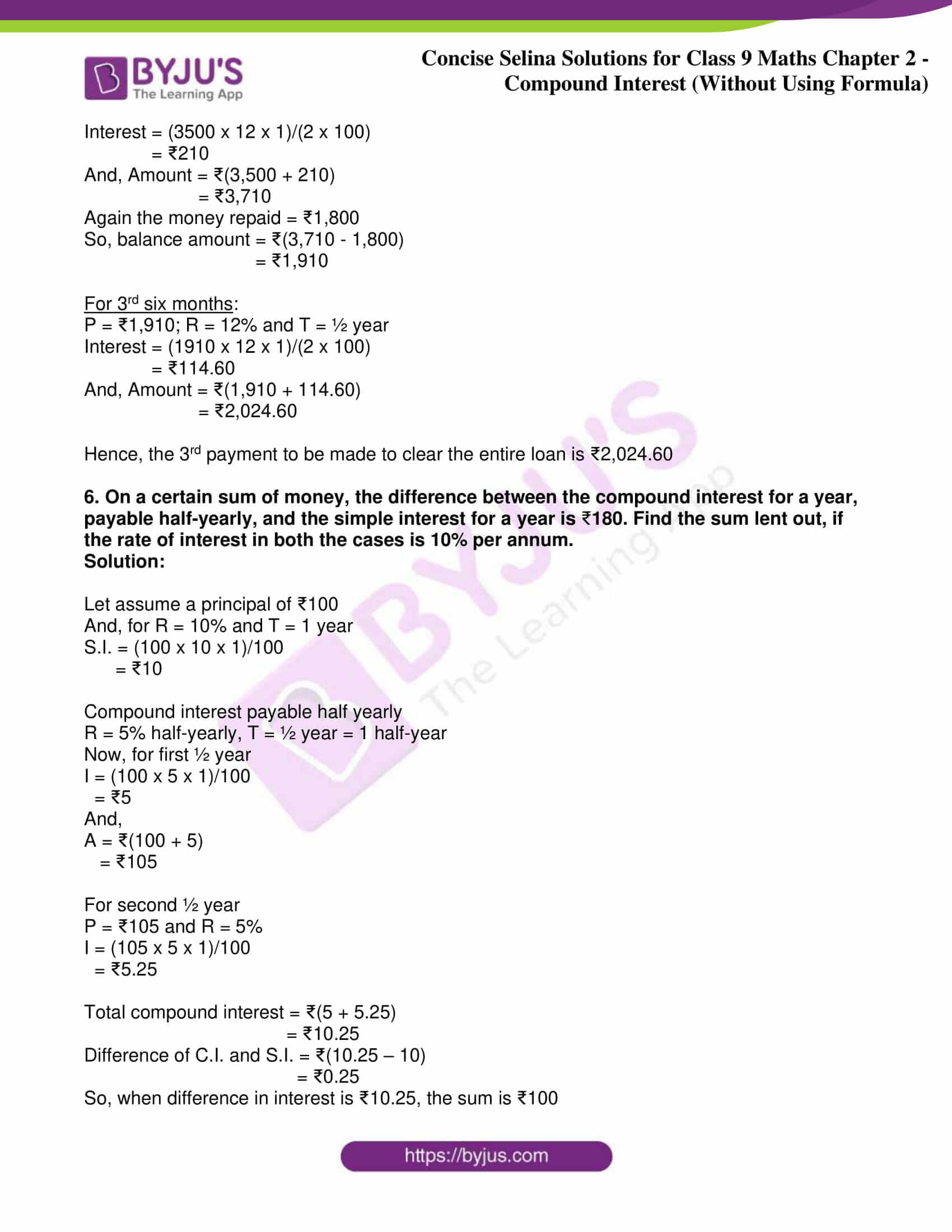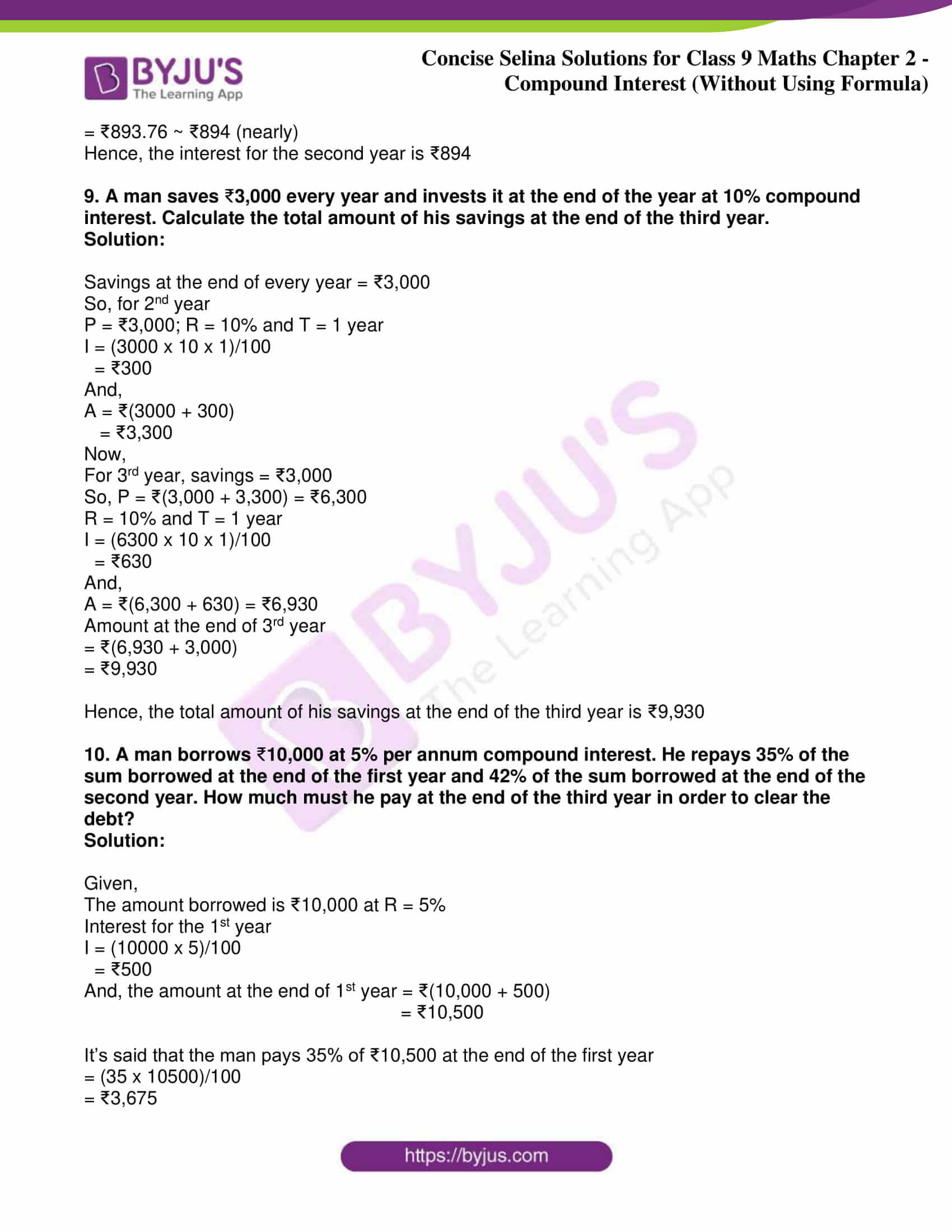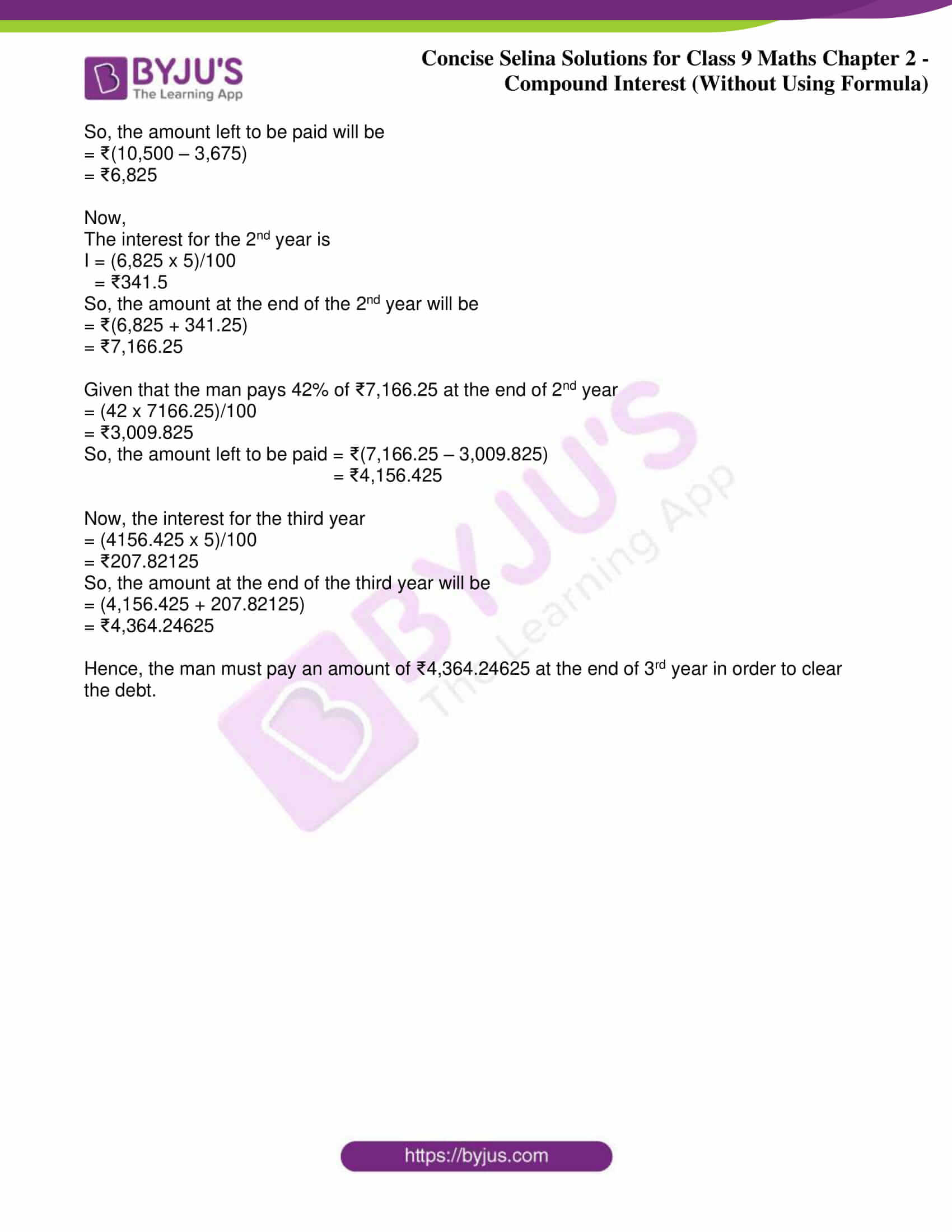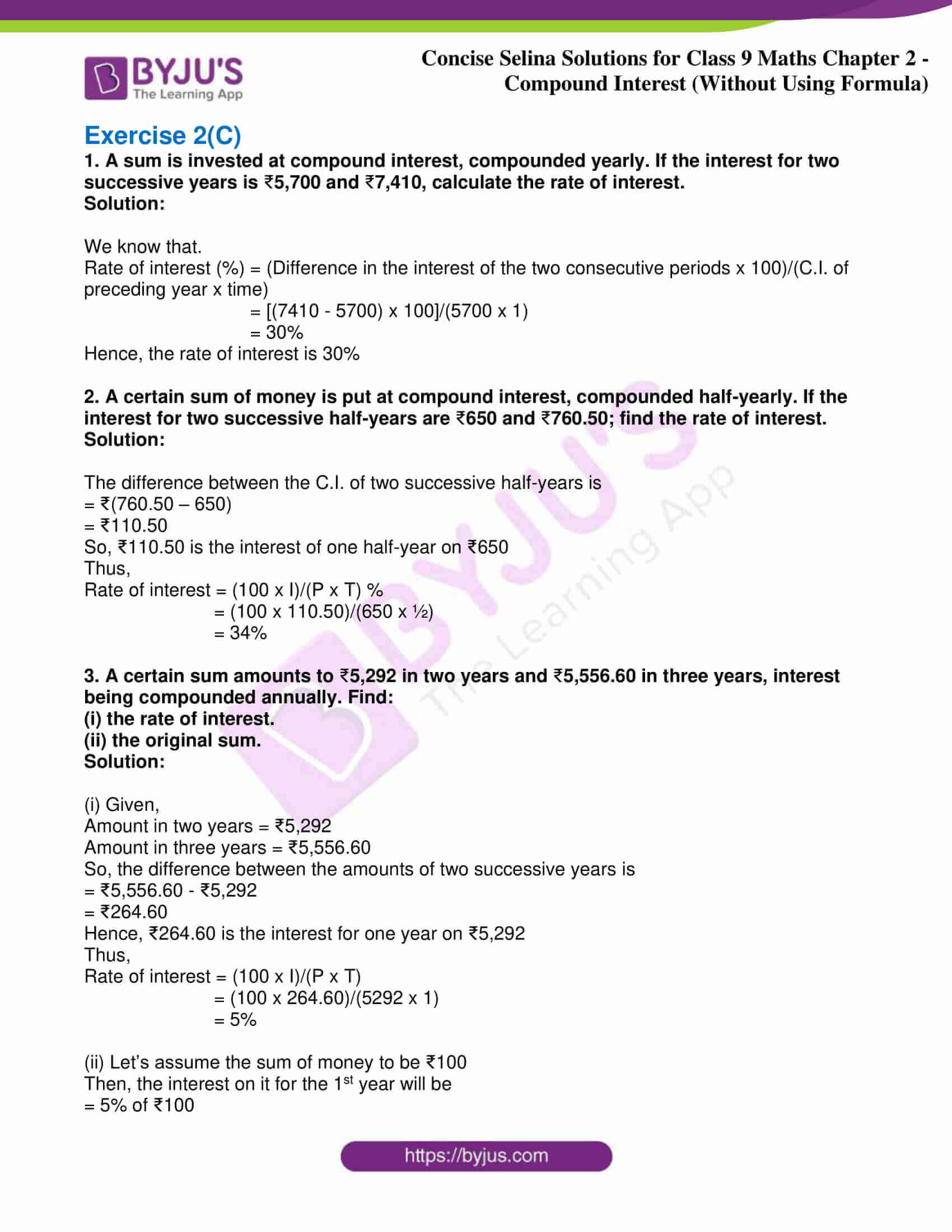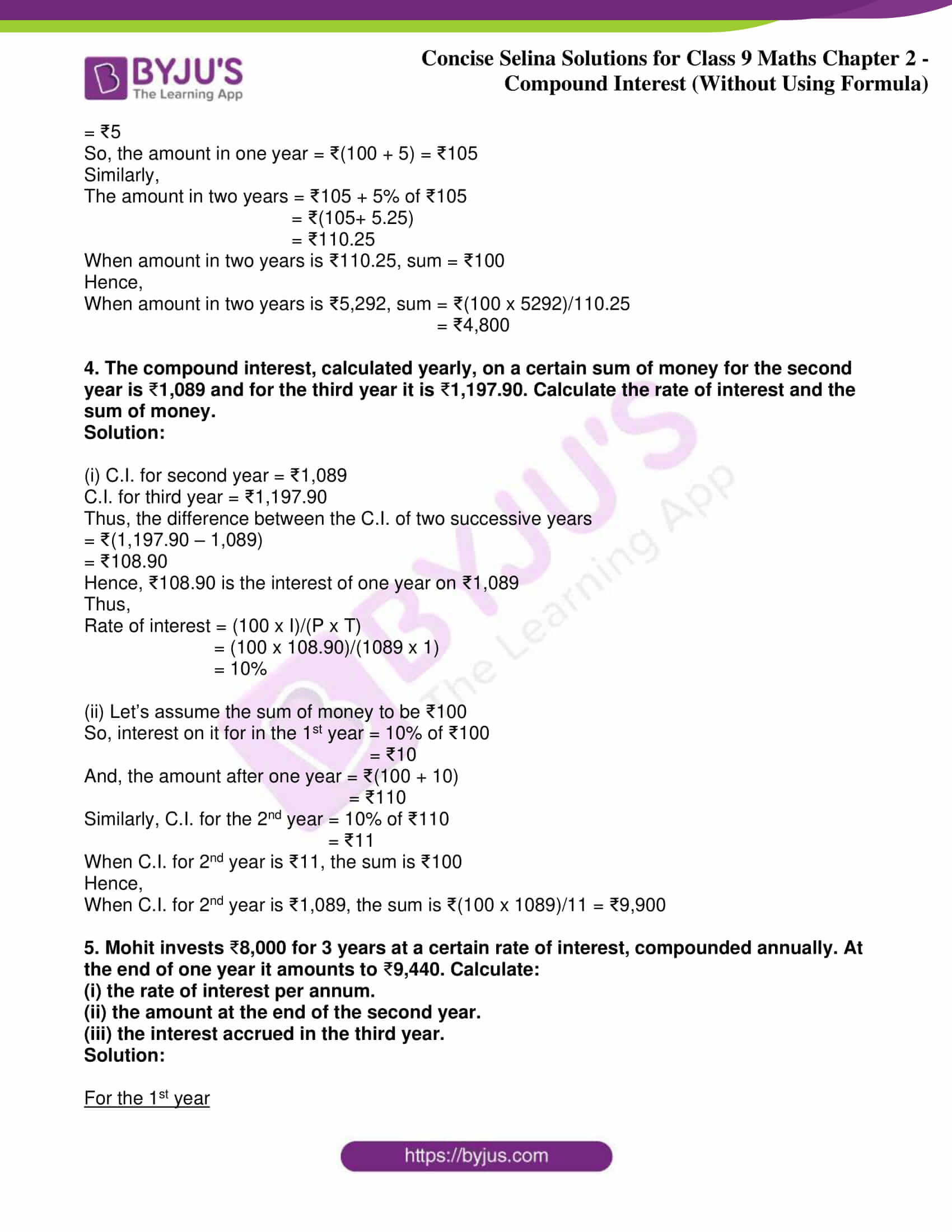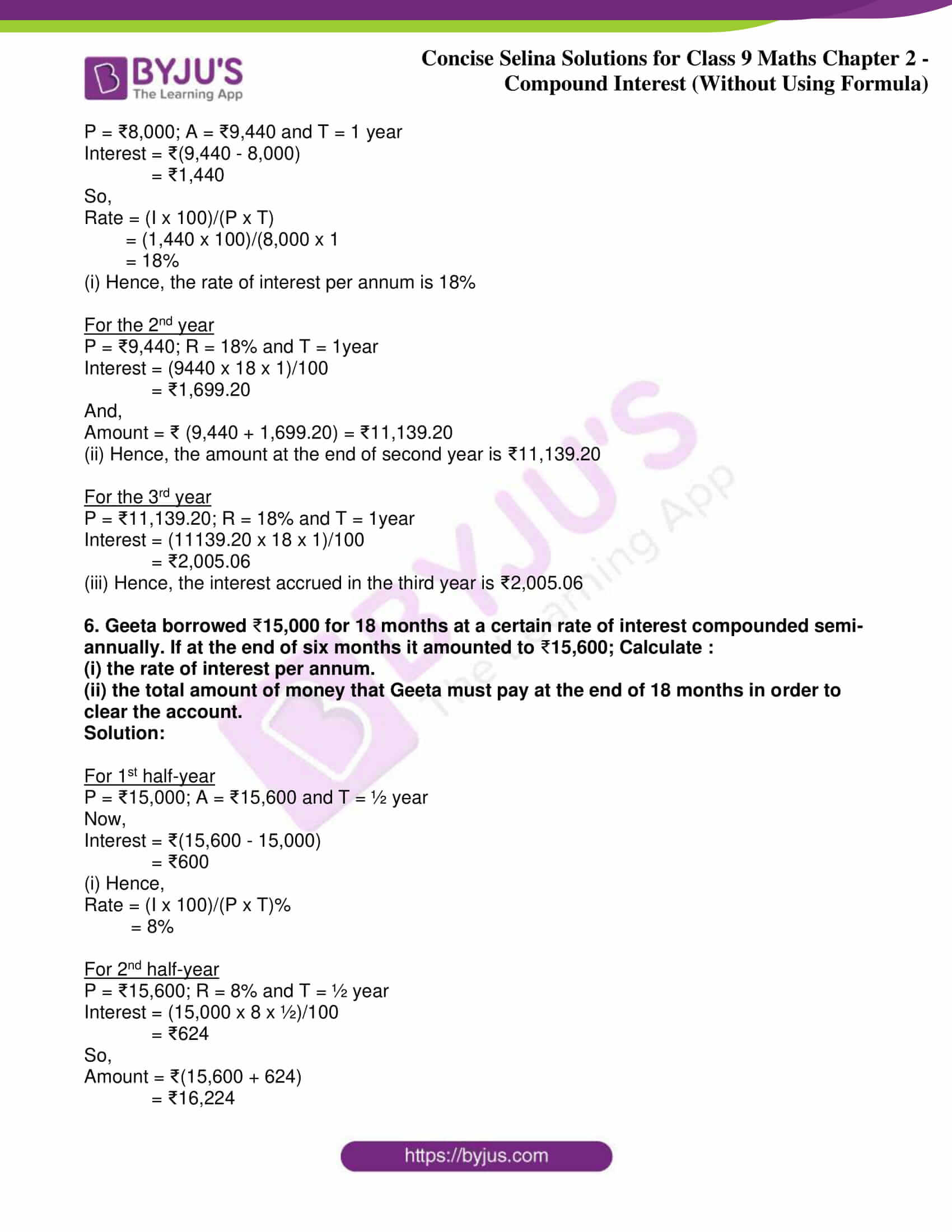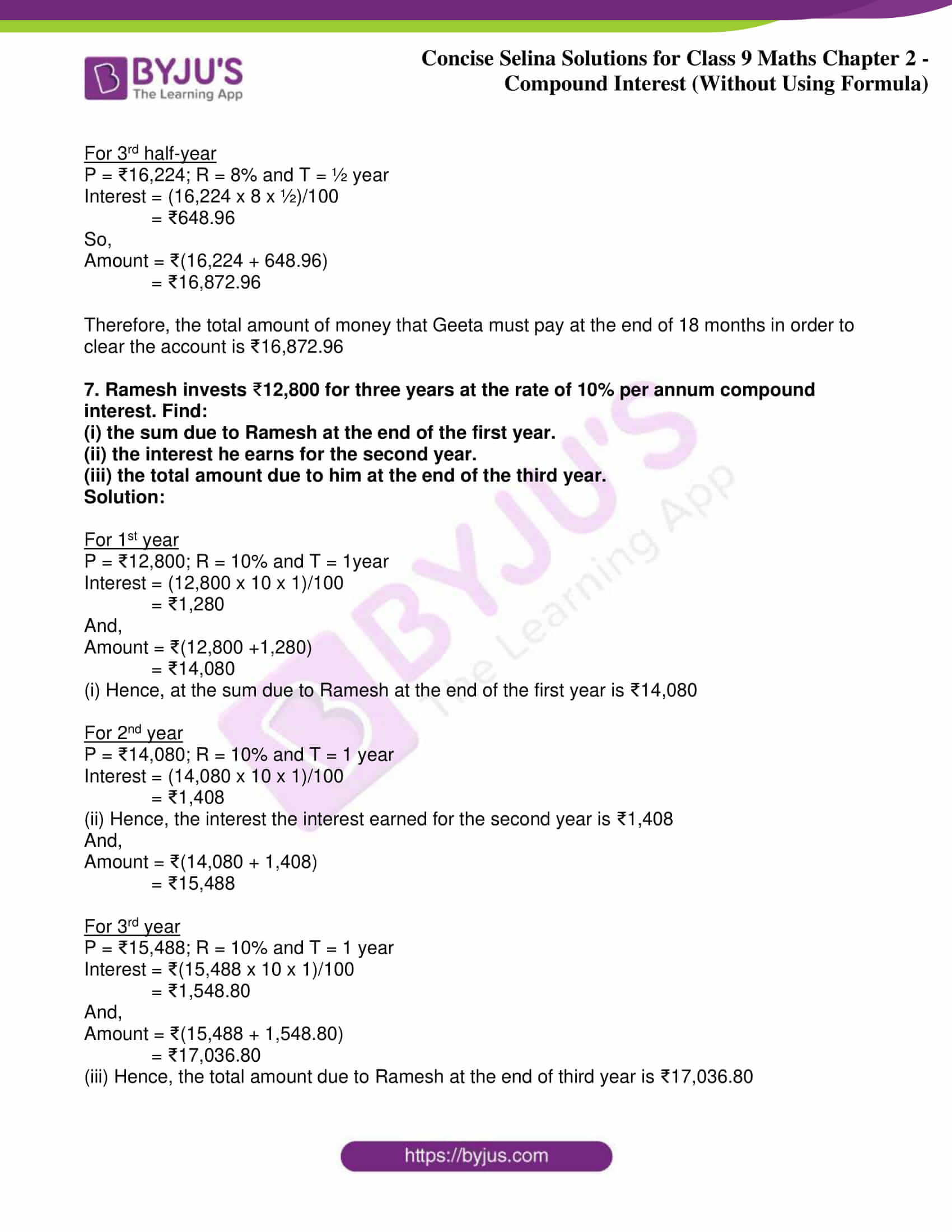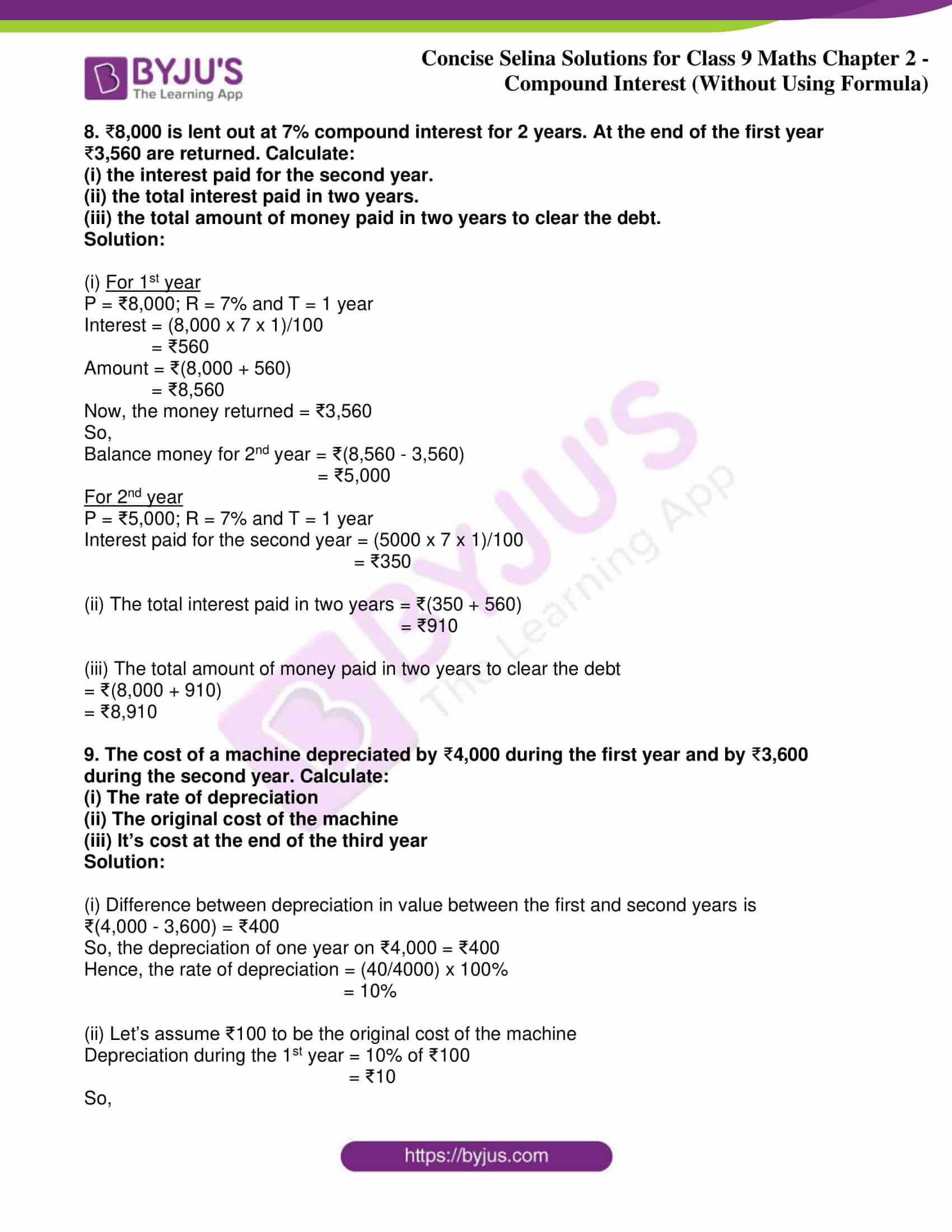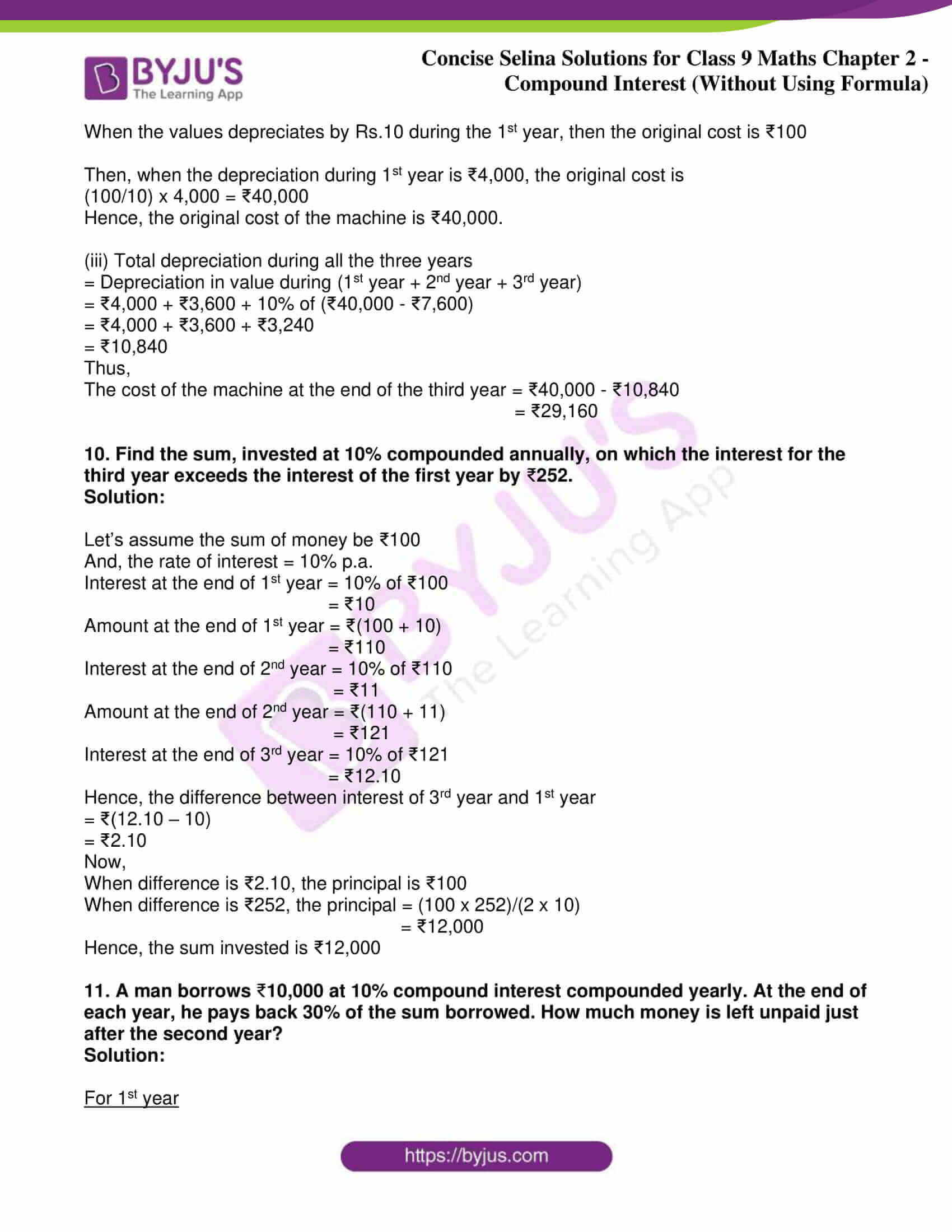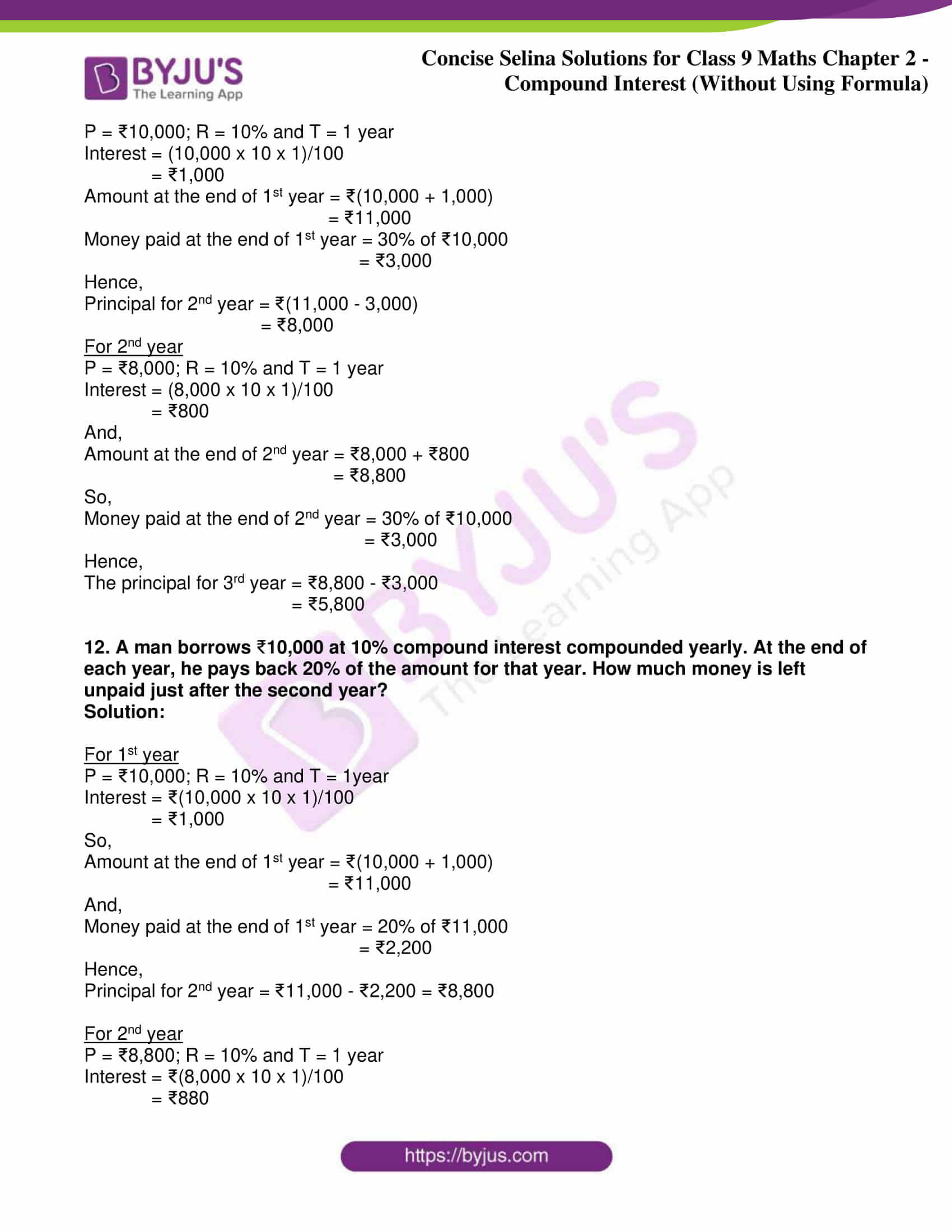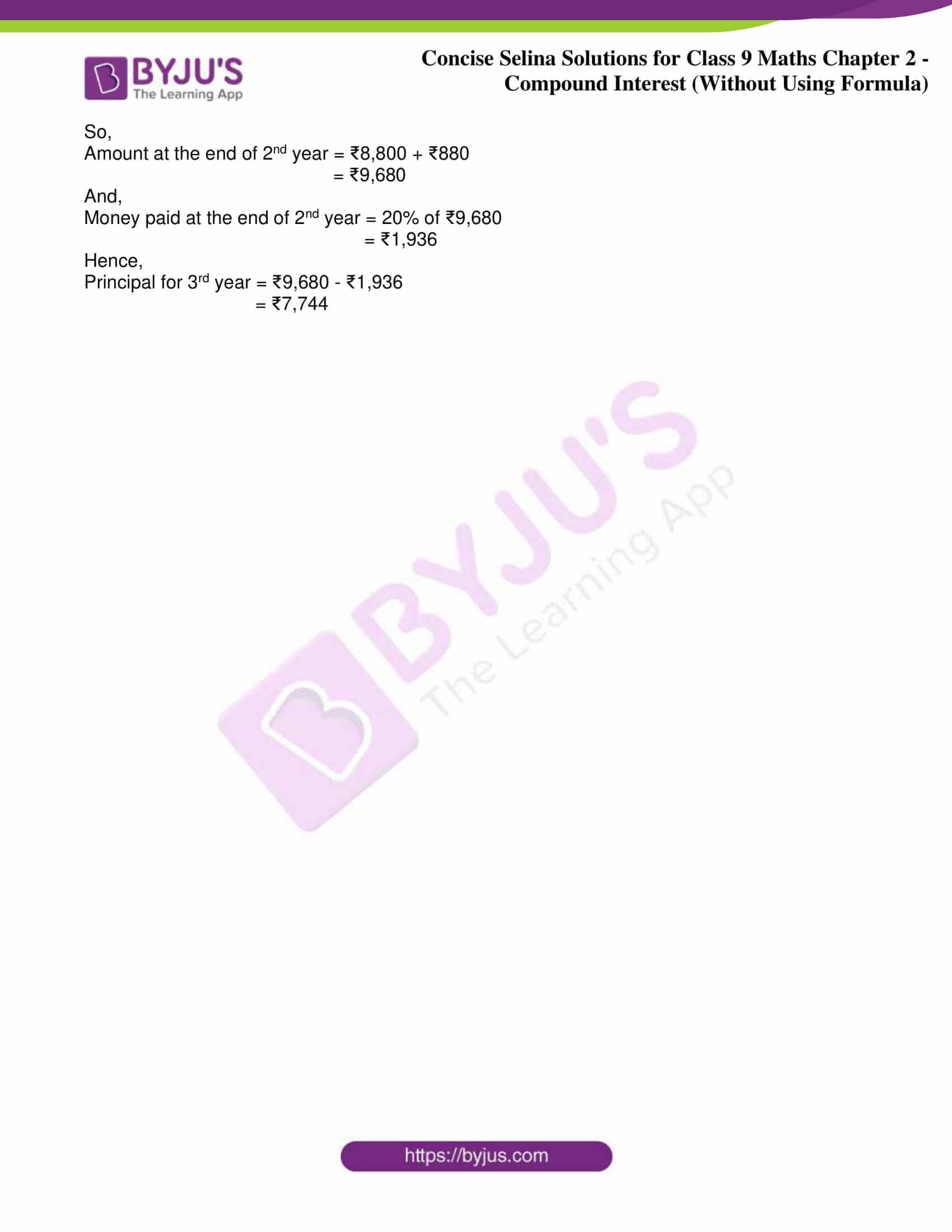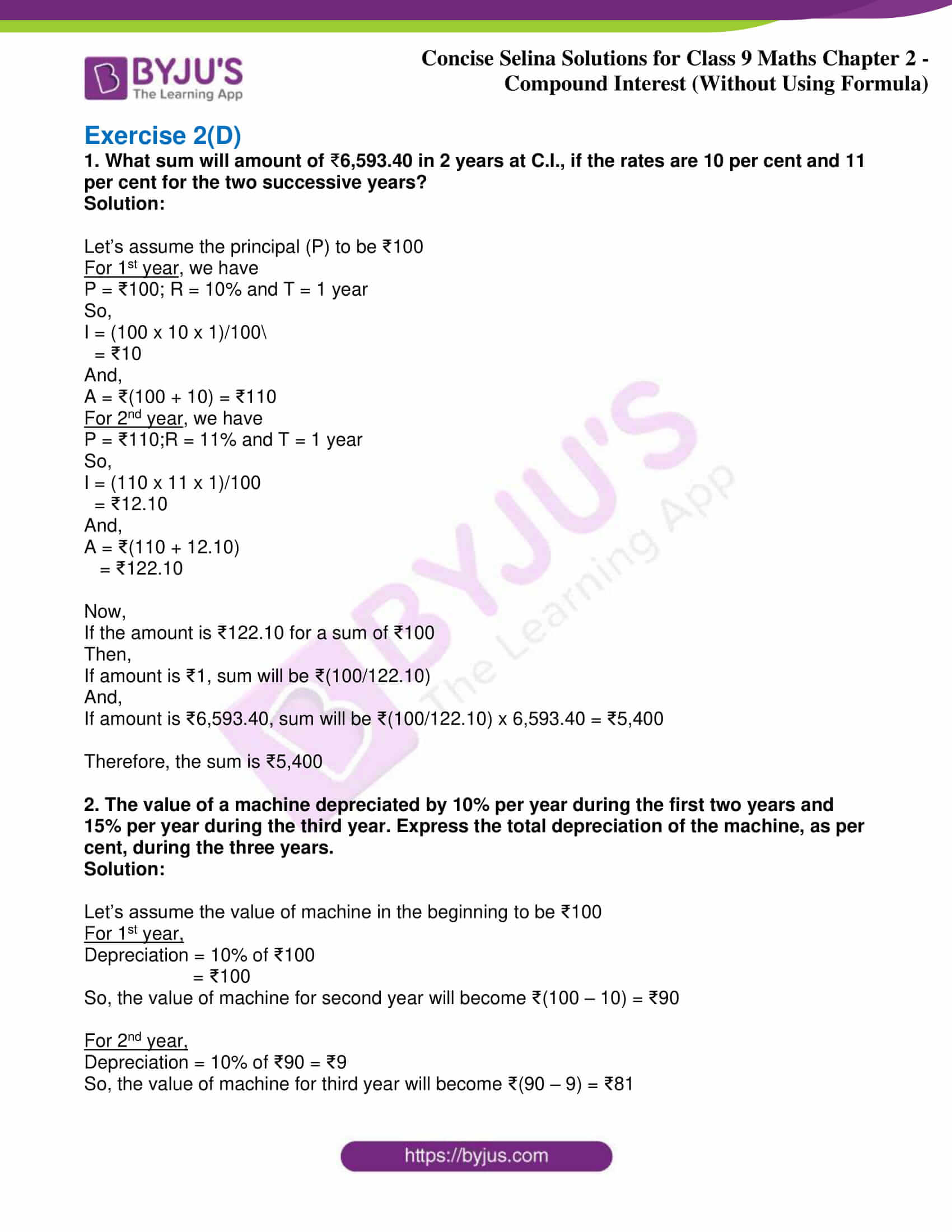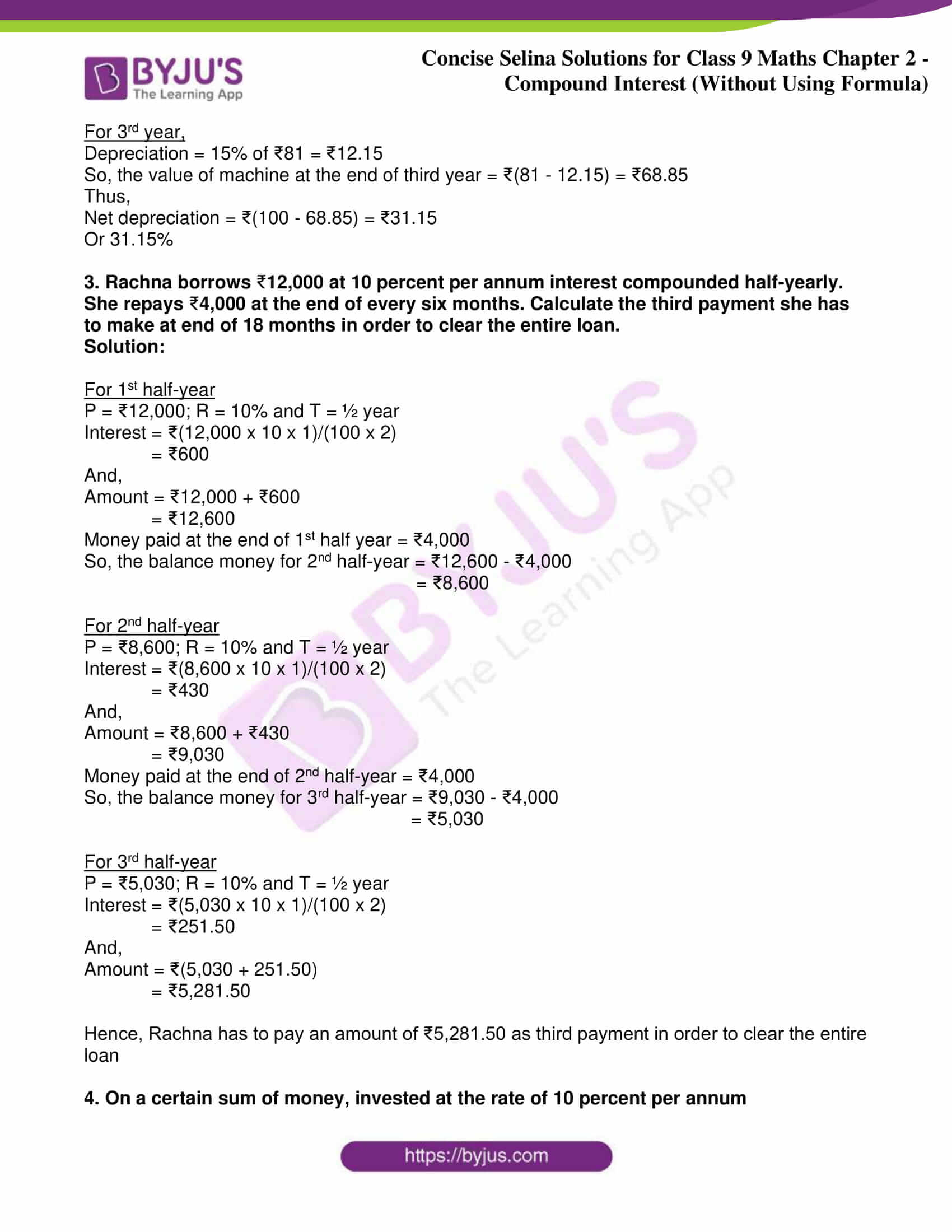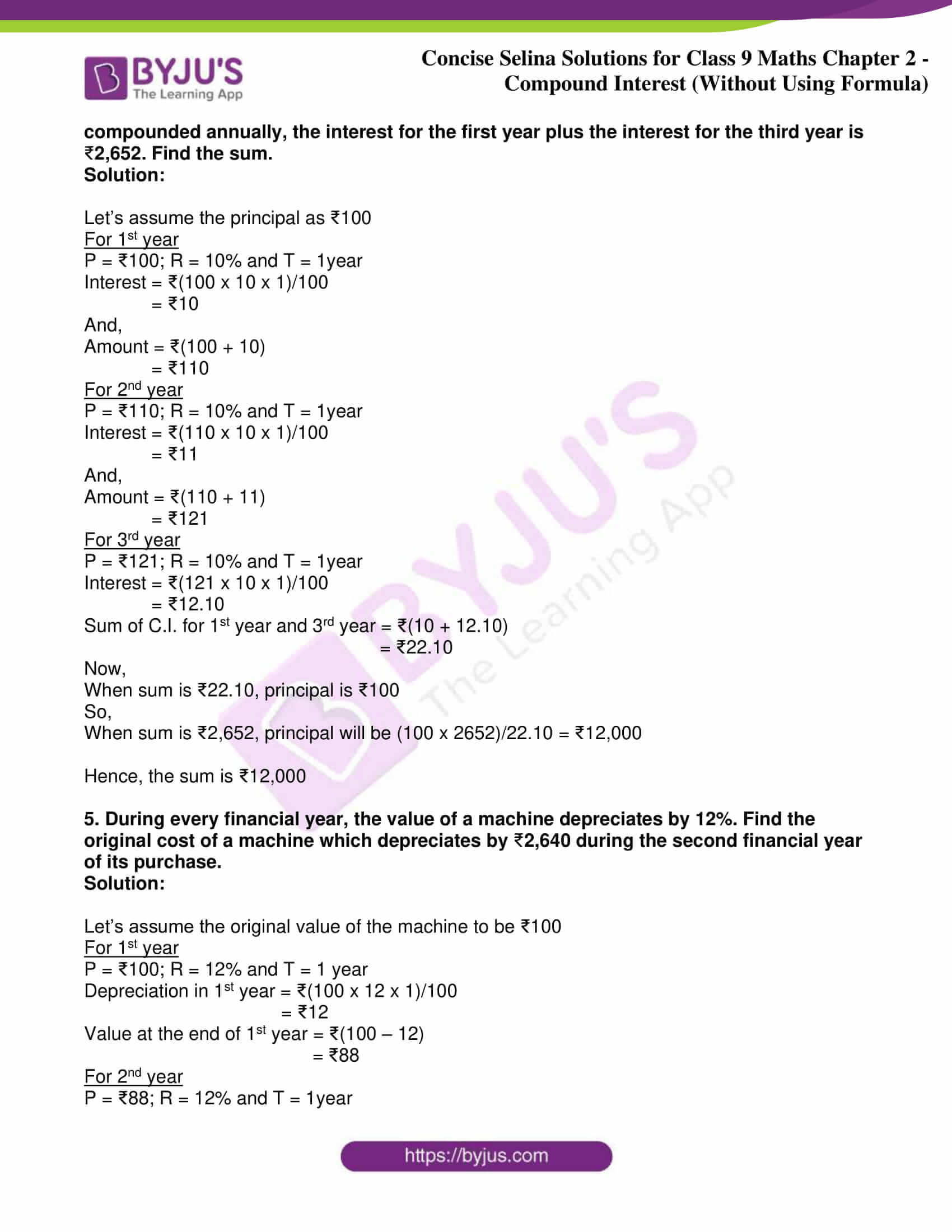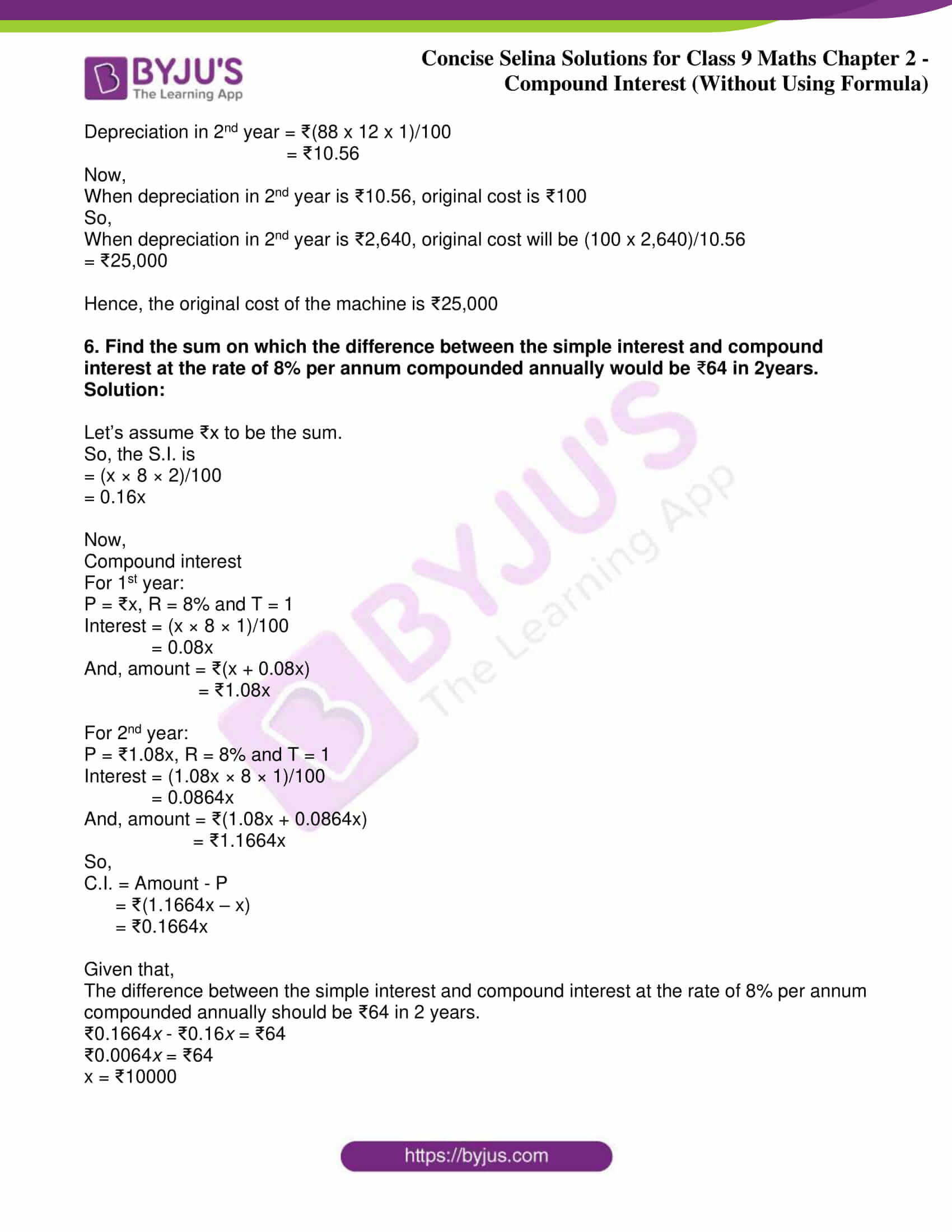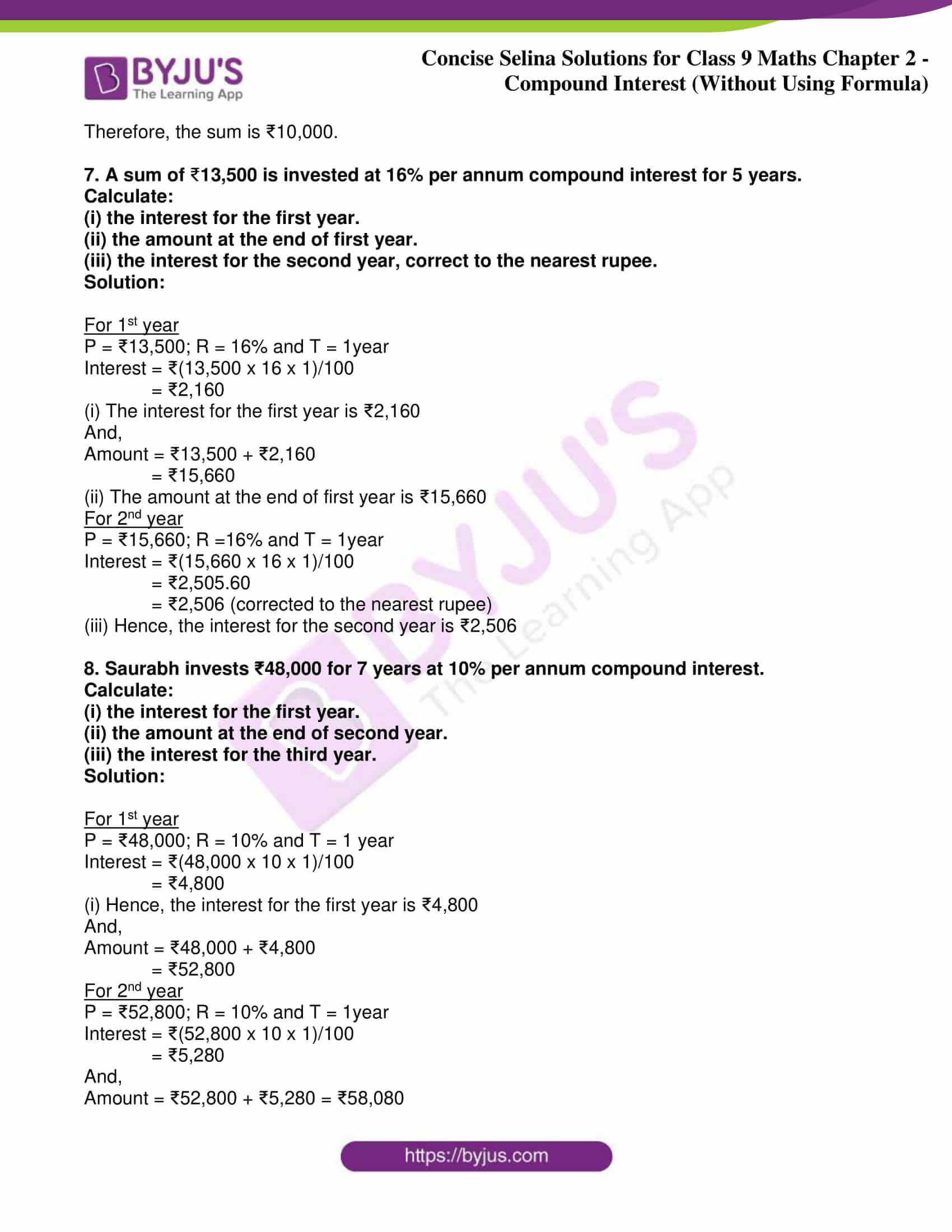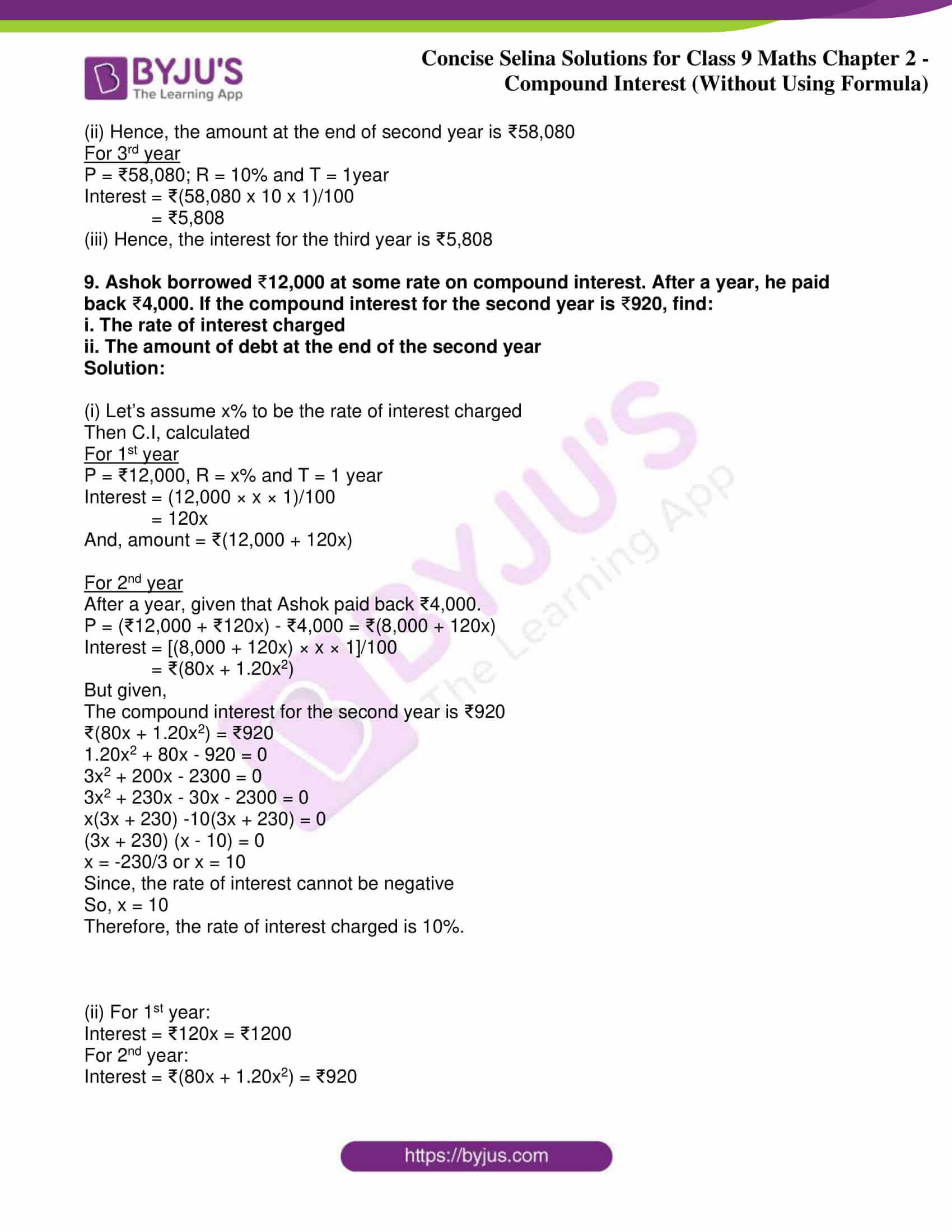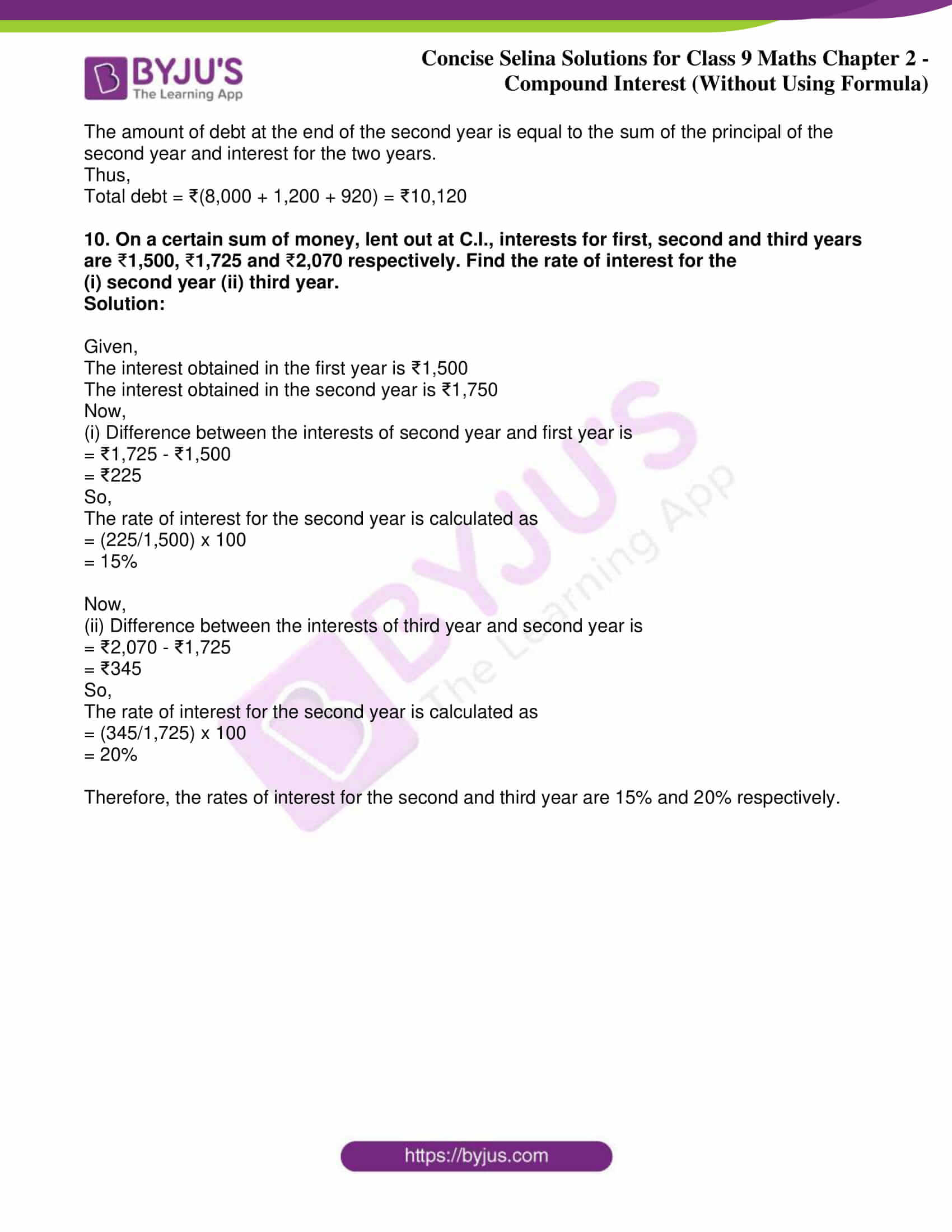### Access Answers of Maths Selina Class 9 Chapter 2- Compound Interest [Without Using Formula]

Exercise 2(A)

1. ₹16,000 is invested at 5% compound interest compounded per annum.

Use the table, given below, to find the amount in 4 years.

 Year Initial amount (₹) Interest (₹) Final amount 1st 16,000 800 16,800 2nd 3rd 4th 5th

Solution:

 Year Initial amount (₹) Interest (₹) Final amount 1st 16,000 800 16,800 2nd 16,800 840 17,640 3rd 17,640 882 18,522 4th 18,522 926.10 19,448.10 5th 19,448.10 972.405 20,420.505

Thus, the amount in 4 years is ₹19,448.10

2.(i) Calculate the amount and the compound interest on:

6000 in 3 years at 5% per year.

(ii) Calculate amount and the compound interest on:

8000 in 2½ years at 15% per annum.

Solution:

(i) Given: P = ₹6,000; N = 3 years and R = 5%

For the 1st year

P = ₹6000; N = 1 year and R = 5%

Interest = (6000 x 5 x 1)/100

= ₹300

And, amount = ₹(6000 + 300)

= ₹6,300

For the 2nd year

P = ₹6300; N = 1 year and R = 5%

Interest = (6300 x 5 x 1)/100

= ₹315

And, amount = ₹(6300 + 315)

= ₹6,615

For the 3rd year

P = ₹6,615; N = 1 year and R = 5%

Interest = (6615 x 5 x 1)/100

= ₹330.75

And, amount = ₹(6,615 + 330.75)

= ₹6,945.75

Hence, the C.I. accrued = Final amount – Initial principal

= ₹6,945.75 – ₹6,000

= ₹945.75

(ii) Given: P = 8000; N = 2½ years and R = 15%

For the 1st year

P = ₹8,000; N = 1 year and R = 15%

Interest = (8000 x 15 x 1)/100

= ₹1,200

And, amount = ₹(8,000 + 1,200)

= ₹9,200

For the 2nd year

P = ₹9,200; N = 1 year and R = 15%

Interest = (9200 x 15 x 1)/100

= ₹1,380

And, amount = ₹(9,200 + 1,380)

= ₹10,580

For the next ½ year

P = ₹10,580; N = ½ year and R = 15%

Interest = (10580 x 15 x ½)/100

= ₹793.50

And, amount = ₹(10,580 + 793.50)

= ₹11,373.50

Hence, the C.I. accrued = Final amount – Initial principal

= ₹11,373.50 – ₹8,000

= ₹3,373.50

3. Calculate the amount and the compound interest on:

(i) 4,600 in 2 years when the rates of interest of successive years are 10% and 12% respectively.

(ii) 6,000 in 3 years, when the rates of the interest for successive years are 10%, 14% and 15% respectively.

Solution:

(i) For 1st year

P = ₹4,600; R = 10% and T = 1 year

I = (4600 x 10 x 1)/100

= ₹460

And,

A = ₹(4,600 + 460)

= ₹5,060

For 2nd year

P = ₹5,060; R = 12% and T = 1 year.

I = (5060 x 12 x1)/100

= 60720/100

= ₹607.20

And,

A = ₹(5,060 + 607.20)

= ₹5,667.20

Compound interest = ₹(5,667.20 – 4,600)

= ₹1,067.20

Amount after 2 years = ₹5,667.20

(ii) For 1st year

P = ₹16,000; R = 10% and T = 1 year

I = (16000 x 10 x 1)/100

= ₹1,600

And,

A = ₹(16,000 + 1,600)

= ₹17,600

For 2nd year

P = ₹17,600; R = 14% and T = 1 year

I = (17600 x 14 x 1)/100

= 246400/100

= ₹2,464

And,

A = ₹(17,600 + 2,464)

= ₹20,064

For 3rd year,

P = ₹20,064; R = 15% and T = 1 year

I = (20064 x 15 x 1)/100

= ₹3,009.60

And,

Amount after 3 years = ₹(20,064 + 3,009.60)

= ₹23,073.60

Hence,

Compound interest = ₹(23,073.60 – 16,000)

= ₹7,073.60

4. Find the compound interest, correct to the nearest rupee, on 2,400 for 2½ years at 5 per cent per annum.

Solution:

For 1st year

P = ₹2400; R = 5% and T = 1 year

I = (2400 x 5 x 1)/100

= ₹120

A = ₹(2400 + 120)

= ₹2520

For 2nd year

P = ₹2520; R = 5% and T = 1 year

I = (2520 x 5 x 1)/100

= ₹126

A = ₹(2,520 + 126)

= ₹2,646

For the final ½ year

P = Rs. 2646; R = 5% and T = ½ year

I = (2646 x 5 x 1)/(100 x 2)

= ₹66.15

Amount after 2½ years = ₹2,646 + ₹66.15

= ₹2,712.15

Hence,

Compound interest = ₹(2,712.15 – 2,400)

= ₹312.15

5. Calculate the compound interest for the second year on 8,000 invested for 3 years at 10% per annum.

Solution:

For 1st year

P = ₹8,000; R = 10% and T = 1 year

I = (8000 x 10 x 1)/100

= 800

And,

A = ₹(8,000 + 800) = ₹8,800

For 2nd year

P = ₹8,800; R = 10% and T = 1 year

I = (8800 x 10 x 1)/100

= ₹880

Hence,

Compound interest for 2nd years = ₹880

6. A borrowed 2,500 from B at 12% per annum compound interest. After 2 years, A gave 2,936 and a watch to B to clear the account. Find the cost of the watch.

Solution:

For 1st year

P = ₹2500; R = 12% and T = 1 year

I = (2500 x 12 x 1)/100

= ₹300

And,

Amount = ₹(2,500 + 300) = ₹2,800

For 2nd year

P = ₹2,800; R = 12% and T = 1 year

I = (2800 x 12 x 1)/100

= ₹336

And,

Amount = ₹(2,800 + 336) = ₹3136

Now,

Amount repaid by A to B = ₹2936

The amount of watch = ₹(3136 – 2936) = ₹200

7. How much will 50,000 amount to in 3 years, compounded yearly, if the rates for the successive years are 6%, 8% and 10% respectively?

Solution:

Given: P = ₹50,000; T = 3 years

Interest for the 1st year, R = 6%

I = (P x R x T)/100

= (50000 x 6 x 1)/100

= ₹3,000

And,

Amount after the 1st year = ₹(3,000 + 50,000)

= ₹53,000

Now,

Interest for the 2nd year, R = 8% and P = ₹53,000

I = (P x R x T)/100

= (53000 x 8 x 1)/100

= ₹4,240

And,

Amount after the 2nd year = ₹(4,240 + 53,000)

= ₹57,240

Next,

Interest for the 3rd year, R = 10% and P = ₹57,240

I = (P x R x T)/100

= (57240 x 10 x 1)/100

= ₹5,724

And,

Amount after the 3rd year = ₹(5,724 + 57,240)

= ₹62,964

Hence, the amount after 3 years will be ₹62,964

8. Meenal lends 75,000 at C.I. for 3 years. If the rate of interest for the first two years is 15% per year and for the third year it is 16%, calculate the sum Meenal will get at the end of the third year.

Solution:

Given: P = ₹75,000; T = 3 years

Interest for the 1st year, R = 15%

I = (P x R x T)/100

= (75000 x 15 x 1)/100

= ₹11,250

And,

Amount after the 1st year = ₹(75,000 + 11,250)

= ₹86,250

Now,

Interest for the 2nd year, R = 15% and P = ₹86,250

I = (P x R x T)/100

= (86250 x 15 x 1)/100

= ₹12,937.50

And,

Amount after the 2nd year = ₹(12,937.50 + 86,250)

= ₹99,187.50

Next,

Interest for the 3rd year, R = 16% and P = ₹99,187.50

I = (P x R x T)/100

= (99187.50 x 16 x 1)/100

= ₹15,870

And,

Amount after the 3rd year = ₹(15,870 + 99,187.50)

= ₹1,15,057.5

Hence, at the end of 3 years Meenal will get an amount of ₹1,15,057.5

9. Govind borrows 18,000 at 10% simple interest. He immediately invests the money borrowed at 10% compound interest compounded half-yearly. How much money does Govind gain in one year?

Solution:

Calculating the simple interest

P = ₹18,000; R = 10% and T = 1year, we have

S.I.= (18000 x 10 x 1)/100

= ₹1,800

Calculating the compound interest (compounded half-yearly)

For 1st half- year

P = ₹18,000; R = 10% and T = ½ year

Interest = (18000 x 10 x 1)/(100 x 2)

= ₹900

So,

Amount = ₹18,000 + ₹900 = ₹18,900

Now,

For 2nd half-year

P = ₹18,900; R = 10% and T = ½ year

Interest = (18,900 x 10 x 1)/(100 x 2)

= ₹945 Rs

So,

Amount = ₹18,900 + ₹945 = ₹19,845

Then,

Compound interest = ₹(19,845 -18,000) = ₹1,845

Therefore,

Govind’s gain = ₹(1,845 – 1,800) = ₹45

10. Find the compound interest on ₹4,000 accrued in three years, when the rate of interest is 8% for the first year and 10% per year for the second and the third years.

Solution:

Given: P = ₹4,000; T = 3 years

Interest for the 1st year, R = 8%

I = (P x R x T)/100

= (4000 x 8 x 1)/100

= ₹320

And,

Amount after the 1st year = ₹(4,000 +320)

= ₹4,320

Now,

Interest for the 2nd year, R = 10% and P = ₹4,320

I = (P x R x T)/100

= (4320 x 10 x 1)/100

= ₹432

And,

Amount after the 2nd year = ₹(432 + 4,320)

= ₹4,752

Next,

Interest for the 3rd year, R = 10% and P = ₹4,752

I = (P x R x T)/100

= (4,752 x 10 x 1)/100

= ₹475.20

And,

Amount after the 3rd year = ₹(475.20 + 4,752)

= ₹5,227.20

Hence,

The compound interest = ₹(5227.20 – 4,000)

= ₹1,227.20

Exercise 2(B)

1. Calculate the difference between the simple interest and the compound interest on 4,000 in 2 years at 8% per annum compounded yearly.

Solution:

For 1st year

P = ₹4,000; R = 8% and T = 1 year

I = (4,000 x 8 x 1)/100

= ₹320

And,

A = ₹(4,000 + 320)

= ₹4,320

For 2nd year

P = ₹4,320; R = 8% and T = 1 year

I = (4,320 x 8 x 1)/100

= ₹345.60

And,

A = ₹(4,320 + 345.60)

= ₹4,665.60

Hence,

Compound interest = ₹(4,665.60 – 4,000)

= ₹665.60

Now,

Simple interest for 2 years = (4000 x 8 x 2)/100

= ₹640

Hence,

Difference of CI and SI = ₹(665.60 – 640)

= ₹25.60

2. A man lends 12,500 at 12% for the first year, at 15% for the second year and at 18% for the third year. If the rates of interest are compounded yearly ; find the difference between the C.I. for the first year and the compound interest for the third year.

Solution:

For 1st year

P = Rs. 12500; R = 12% and R = 1 year

I = (12500 x 12 x 1)/100

= ₹1,500

And,

A = ₹(12,500 + 1,500)

= ₹14,000

For 2nd year

P = ₹14,000; R = 15% and T = 1 year

I = (14000 x 15 x 1)/100

= ₹2,100

And,

A = ₹(1,400 + 2,100)

= ₹16,100

For 3rd year

P = ₹16,100; R = 18% and T = 1 year

I = (16100 x 18 x 1)/100

= ₹2898

And,

A = ₹(16,100 + 2,898)

= ₹18,998

Hence,

The difference between the compound interest of the third year and first year

= ₹2,898 – ₹1,500

= ₹1,398

3. A sum of money is lent at 8% per annum compound interest. If the interest for the second year exceeds that for the first year by 96, find the sum of money.

Solution:

Let’s assume the money lent to be ₹100

So,

For 1st year

P = ₹100; R = 8% and T = 1 year

Interest for the first year = (100 x 8 x 1)/100

= ₹8

Amount = ₹(100+ 8)

= ₹108

For 2nd year

P = ₹108; R = 8% and T = 1year

Interest for the second year = (108 x 8 x 1)/100

= ₹8.64

Now,

Difference between the interests for the second and first year = ₹(8.64 – 8) = ₹0.64

But given that interest for the second year exceeds the first year by ₹96

Then,

When the difference between the interests is ₹0.64, principal is ₹100

So,

When the difference between the interests is ₹96, principal = ₹(96 x 100/0.64)

= ₹15,000

Therefore, the sum of money lent is ₹15,000

4. A man borrows 6,000 at 5% C.I. per annum. If he repays 1,200 at the end of each year, find the amount of the loan outstanding at the beginning of the third year.

Solution:

Given, amount borrowed = ₹6,000 at R = 5% C.I. per annum

So,

Interest for the 1st year = (5/100 x 6000)

= ₹300

And, the amount at the end of the first year will be

= ₹(6,000 + 300)

= ₹6,300

Given that an amount of ₹1,200 is repaid at the end of each year

Now,

The amount left to the paid at the end of 1st year

= ₹(6,300 – 1,200)

= ₹5,100

Then, the interest for the 2nd year is

= (5/100 x 5100)

= ₹255

And, the amount will be = ₹(5100 + 255)

= ₹5,355

Now, the amount left to be paid at the end of 2nd year after reduction of ₹1,200 will be

= ₹(5,355 – 1,200)

= ₹4,155

Hence, the amount of the loan outstanding at the beginning of the third year is ₹4,155

5. A man borrows 5,000 at 12 percent compound interest payable every six months. He repays 1,800 at the end of every six months. Calculate the third payment he has to make at the end of 18 months in order to clear the entire loan.

Solution:

For 1st six months:

P = ₹5,000; R = 12% and T = ½ year

Interest = (5000 x 12 x 1)/(2 x 100)

= ₹300

And, Amount = ₹(5,000 + 300)

= ₹5,300

Given that the money repaid = ₹1,800

So, balance amount = ₹(5,300 – 1,800)

= ₹3,500

For 2nd six months:

P = ₹3,500; R = 12% and T = ½ year

Interest = (3500 x 12 x 1)/(2 x 100)

= ₹210

And, Amount = ₹(3,500 + 210)

= ₹3,710

Again the money repaid = ₹1,800

So, balance amount = ₹(3,710 – 1,800)

= ₹1,910

For 3rd six months:

P = ₹1,910; R = 12% and T = ½ year

Interest = (1910 x 12 x 1)/(2 x 100)

= ₹114.60

And, Amount = ₹(1,910 + 114.60)

= ₹2,024.60

Hence, the 3rd payment to be made to clear the entire loan is ₹2,024.60

6. On a certain sum of money, the difference between the compound interest for a year, payable half-yearly, and the simple interest for a year is 180. Find the sum lent out, if the rate of interest in both the cases is 10% per annum.

Solution:

Let assume a principal of ₹100

And, for R = 10% and T = 1 year

S.I. = (100 x 10 x 1)/100

= ₹10

Compound interest payable half yearly

R = 5% half-yearly, T = ½ year = 1 half-year

Now, for first ½ year

I = (100 x 5 x 1)/100

= ₹5

And,

A = ₹(100 + 5)

= ₹105

For second ½ year

P = ₹105 and R = 5%

I = (105 x 5 x 1)/100

= ₹5.25

Total compound interest = ₹(5 + 5.25)

= ₹10.25

Difference of C.I. and S.I. = ₹(10.25 – 10)

= ₹0.25

So, when difference in interest is ₹10.25, the sum is ₹100

So, if the difference is ₹1, the sum is (100/0.25) = 400

And,

If the difference is ₹180, the sum will be ₹(400 x 180) =  ₹72,000

Hence, the sum lent out is ₹72,000

7. A manufacturer estimates that his machine depreciates by 15% of its value at the beginning of the year. Find the original value (cost) of the machine, if it depreciates by 5,355 during the second year.

Solution:

Let’s assume the original cost of the machine to be ₹100

Given that the machine depreciates by 15% during the first year

So, 15% of ₹100 = ₹15

Now,

The value of the machine at the beginning of the 2nd year will be

= ₹(100 – 15)

= ₹85

Again, the depreciation during the 2nd year = 15% of ₹85 = ₹12.75

Now,

When the depreciation during the 2nd year is ₹12.75, the original cost is ₹100

So,

When the depreciation during the 2nd year is ₹5,355, the original cost will be

= (100 x 5355)/12.75

= ₹42,000

Therefore, the original cost of the machine is ₹42,000

8. A man invest 5,600 at 14% per annum compound interest for 2 years. Calculate:

(i) The interest for the first year.

(ii) The amount at the end of the first year.

(iii) The interest for the second year, correct to the nearest rupee.

Solution:

(i) For the 1st year

P = ₹5,600; R = 14% and T = 1 year

I = (5600 x 14 x 1)/100

= ₹784

And,

(ii) Amount at the end of the first year is

= ₹(5600 + 784)

= ₹6,384

(iii) Now, for the 2nd year

P = ₹6,384; R = 14% and R = 1 year

I = (6384 x 14 x 1)/100

= ₹893.76 ~ ₹894 (nearly)

Hence, the interest for the second year is ₹894

9. A man saves 3,000 every year and invests it at the end of the year at 10% compound interest. Calculate the total amount of his savings at the end of the third year.

Solution:

Savings at the end of every year = ₹3,000

So, for 2nd year

P = ₹3,000; R = 10% and T = 1 year

I = (3000 x 10 x 1)/100

= ₹300

And,

A = ₹(3000 + 300)

= ₹3,300

Now,

For 3rd year, savings = ₹3,000

So, P = ₹(3,000 + 3,300) = ₹6,300

R = 10% and T = 1 year

I = (6300 x 10 x 1)/100

= ₹630

And,

A = ₹(6,300 + 630) = ₹6,930

Amount at the end of 3rd year

= ₹(6,930 + 3,000)

= ₹9,930

Hence, the total amount of his savings at the end of the third year is ₹9,930

10. A man borrows 10,000 at 5% per annum compound interest. He repays 35% of the sum borrowed at the end of the first year and 42% of the sum borrowed at the end of the second year. How much must he pay at the end of the third year in order to clear the debt?

Solution:

Given,

The amount borrowed is ₹10,000 at R = 5%

Interest for the 1st year

I = (10000 x 5)/100

= ₹500

And, the amount at the end of 1st year = ₹(10,000 + 500)

= ₹10,500

It’s said that the man pays 35% of ₹10,500 at the end of the first year

= (35 x 10500)/100

= ₹3,675

So, the amount left to be paid will be

= ₹(10,500 – 3,675)

= ₹6,825

Now,

The interest for the 2nd year is

I = (6,825 x 5)/100

= ₹341.5

So, the amount at the end of the 2nd year will be

= ₹(6,825 + 341.25)

= ₹7,166.25

Given that the man pays 42% of ₹7,166.25 at the end of 2nd year

= (42 x 7166.25)/100

= ₹3,009.825

So, the amount left to be paid = ₹(7,166.25 – 3,009.825)

= ₹4,156.425

Now, the interest for the third year

= (4156.425 x 5)/100

= ₹207.82125

So, the amount at the end of the third year will be

= (4,156.425 + 207.82125)

= ₹4,364.24625

Hence, the man must pay an amount of ₹4,364.24625 at the end of 3rd year in order to clear the debt.

Exercise 2(C)

1. A sum is invested at compound interest, compounded yearly. If the interest for two successive years is 5,700 and 7,410, calculate the rate of interest.

Solution:

We know that.

Rate of interest (%) = (Difference in the interest of the two consecutive periods x 100)/(C.I. of preceding year x time)

= [(7410 – 5700) x 100]/(5700 x 1)

= 30%

Hence, the rate of interest is 30%

2. A certain sum of money is put at compound interest, compounded half-yearly. If the interest for two successive half-years are 650 and 760.50; find the rate of interest.

Solution:

The difference between the C.I. of two successive half-years is

= ₹(760.50 – 650)

= ₹110.50

So, ₹110.50 is the interest of one half-year on ₹650

Thus,

Rate of interest = (100 x I)/(P x T) %

= (100 x 110.50)/(650 x ½)

= 34%

3. A certain sum amounts to 5,292 in two years and 5,556.60 in three years, interest being compounded annually. Find:

(i) the rate of interest.

(ii) the original sum.

Solution:

(i) Given,

Amount in two years = ₹5,292

Amount in three years = ₹5,556.60

So, the difference between the amounts of two successive years is

= ₹5,556.60 – ₹5,292

= ₹264.60

Hence, ₹264.60 is the interest for one year on ₹5,292

Thus,

Rate of interest = (100 x I)/(P x T)

= (100 x 264.60)/(5292 x 1)

= 5%

(ii) Let’s assume the sum of money to be ₹100

Then, the interest on it for the 1st year will be

= 5% of ₹100

= ₹5

So, the amount in one year = ₹(100 + 5) = ₹105

Similarly,

The amount in two years = ₹105 + 5% of ₹105

= ₹(105+ 5.25)

= ₹110.25

When amount in two years is ₹110.25, sum = ₹100

Hence,

When amount in two years is ₹5,292, sum = ₹(100 x 5292)/110.25

= ₹4,800

4. The compound interest, calculated yearly, on a certain sum of money for the second year is 1,089 and for the third year it is 1,197.90. Calculate the rate of interest and the sum of money.

Solution:

(i) C.I. for second year = ₹1,089

C.I. for third year = ₹1,197.90

Thus, the difference between the C.I. of two successive years

= ₹(1,197.90 – 1,089)

= ₹108.90

Hence, ₹108.90 is the interest of one year on ₹1,089

Thus,

Rate of interest = (100 x I)/(P x T)

= (100 x 108.90)/(1089 x 1)

= 10%

(ii) Let’s assume the sum of money to be ₹100

So, interest on it for in the 1st year = 10% of ₹100

= ₹10

And, the amount after one year = ₹(100 + 10)

= ₹110

Similarly, C.I. for the 2nd year = 10% of ₹110

= ₹11

When C.I. for 2nd year is ₹11, the sum is ₹100

Hence,

When C.I. for 2nd year is ₹1,089, the sum is ₹(100 x 1089)/11 = ₹9,900

5. Mohit invests 8,000 for 3 years at a certain rate of interest, compounded annually. At the end of one year it amounts to 9,440. Calculate:

(i) the rate of interest per annum.

(ii) the amount at the end of the second year.

(iii) the interest accrued in the third year.

Solution:

For the 1st year

P = ₹8,000; A = ₹9,440 and T = 1 year

Interest = ₹(9,440 – 8,000)

= ₹1,440

So,

Rate = (I x 100)/(P x T)

= (1,440 x 100)/(8,000 x 1

= 18%

(i) Hence, the rate of interest per annum is 18%

For the 2nd year

P = ₹9,440; R = 18% and T = 1year

Interest = (9440 x 18 x 1)/100

= ₹1,699.20

And,

Amount = ₹ (9,440 + 1,699.20) = ₹11,139.20

(ii) Hence, the amount at the end of second year is ₹11,139.20

For the 3rd year

P = ₹11,139.20; R = 18% and T = 1year

Interest = (11139.20 x 18 x 1)/100

= ₹2,005.06

(iii) Hence, the interest accrued in the third year is ₹2,005.06

6. Geeta borrowed 15,000 for 18 months at a certain rate of interest compounded semi-annually. If at the end of six months it amounted to 15,600; Calculate :

(i) the rate of interest per annum.

(ii) the total amount of money that Geeta must pay at the end of 18 months in order to clear the account.

Solution:

For 1st half-year

P = ₹15,000; A = ₹15,600 and T = ½ year

Now,

Interest = ₹(15,600 – 15,000)

= ₹600

(i) Hence,

Rate = (I x 100)/(P x T)%

= 8%

For 2nd half-year

P = ₹15,600; R = 8% and T = ½ year

Interest = (15,000 x 8 x ½)/100

= ₹624

So,

Amount = ₹(15,600 + 624)

= ₹16,224

For 3rd half-year

P = ₹16,224; R = 8% and T = ½ year

Interest = (16,224 x 8 x ½)/100

= ₹648.96

So,

Amount = ₹(16,224 + 648.96)

= ₹16,872.96

Therefore, the total amount of money that Geeta must pay at the end of 18 months in order to clear the account is ₹16,872.96

7. Ramesh invests 12,800 for three years at the rate of 10% per annum compound interest. Find:

(i) the sum due to Ramesh at the end of the first year.

(ii) the interest he earns for the second year.

(iii) the total amount due to him at the end of the third year.

Solution:

For 1st year

P = ₹12,800; R = 10% and T = 1year

Interest = (12,800 x 10 x 1)/100

= ₹1,280

And,

Amount = ₹(12,800 +1,280)

= ₹14,080

(i) Hence, at the sum due to Ramesh at the end of the first year is ₹14,080

For 2nd year

P = ₹14,080; R = 10% and T = 1 year

Interest = (14,080 x 10 x 1)/100

= ₹1,408

(ii) Hence, the interest the interest earned for the second year is ₹1,408

And,

Amount = ₹(14,080 + 1,408)

= ₹15,488

For 3rd year

P = ₹15,488; R = 10% and T = 1 year

Interest = ₹(15,488 x 10 x 1)/100

= ₹1,548.80

And,

Amount = ₹(15,488 + 1,548.80)

= ₹17,036.80

(iii) Hence, the total amount due to Ramesh at the end of third year is ₹17,036.80

8. 8,000 is lent out at 7% compound interest for 2 years. At the end of the first year 3,560 are returned. Calculate:

(i) the interest paid for the second year.

(ii) the total interest paid in two years.

(iii) the total amount of money paid in two years to clear the debt.

Solution:

(i) For 1st year

P = ₹8,000; R = 7% and T = 1 year

Interest = (8,000 x 7 x 1)/100

= ₹560

Amount = ₹(8,000 + 560)

= ₹8,560

Now, the money returned = ₹3,560

So,

Balance money for 2nd year = ₹(8,560 – 3,560)

= ₹5,000

For 2nd year

P = ₹5,000; R = 7% and T = 1 year

Interest paid for the second year = (5000 x 7 x 1)/100

= ₹350

(ii) The total interest paid in two years = ₹(350 + 560)

= ₹910

(iii) The total amount of money paid in two years to clear the debt

= ₹(8,000 + 910)

= ₹8,910

9. The cost of a machine depreciated by 4,000 during the first year and by 3,600 during the second year. Calculate:

(i) The rate of depreciation

(ii) The original cost of the machine

(iii) It’s cost at the end of the third year

Solution:

(i) Difference between depreciation in value between the first and second years is

₹(4,000 – 3,600) = ₹400

So, the depreciation of one year on ₹4,000 = ₹400

Hence, the rate of depreciation = (40/4000) x 100%

= 10%

(ii) Let’s assume ₹100 to be the original cost of the machine

Depreciation during the 1st year = 10% of ₹100

= ₹10

So,

When the values depreciates by Rs.10 during the 1st year, then the original cost is ₹100

Then, when the depreciation during 1st year is ₹4,000, the original cost is

(100/10) x 4,000 = ₹40,000

Hence, the original cost of the machine is ₹40,000.

(iii) Total depreciation during all the three years

= Depreciation in value during (1st year + 2nd year + 3rd year)

= ₹4,000 + ₹3,600 + 10% of (₹40,000 – ₹7,600)

= ₹4,000 + ₹3,600 + ₹3,240

= ₹10,840

Thus,

The cost of the machine at the end of the third year = ₹40,000 – ₹10,840

= ₹29,160

10. Find the sum, invested at 10% compounded annually, on which the interest for the third year exceeds the interest of the first year by 252.

Solution:

Let’s assume the sum of money be ₹100

And, the rate of interest = 10% p.a.

Interest at the end of 1st year = 10% of ₹100

= ₹10

Amount at the end of 1st year = ₹(100 + 10)

= ₹110

Interest at the end of 2nd year = 10% of ₹110

= ₹11

Amount at the end of 2nd year = ₹(110 + 11)

= ₹121

Interest at the end of 3rd year = 10% of ₹121

= ₹12.10

Hence, the difference between interest of 3rd year and 1st year

= ₹(12.10 – 10)

= ₹2.10

Now,

When difference is ₹2.10, the principal is ₹100

When difference is ₹252, the principal = (100 x 252)/(2 x 10)

= ₹12,000

Hence, the sum invested is ₹12,000

11. A man borrows 10,000 at 10% compound interest compounded yearly. At the end of each year, he pays back 30% of the sum borrowed. How much money is left unpaid just after the second year?

Solution:

For 1st year

P = ₹10,000; R = 10% and T = 1 year

Interest = (10,000 x 10 x 1)/100

= ₹1,000

Amount at the end of 1st year = ₹(10,000 + 1,000)

= ₹11,000

Money paid at the end of 1st year = 30% of ₹10,000

= ₹3,000

Hence,

Principal for 2nd year = ₹(11,000 – 3,000)

= ₹8,000

For 2nd year

P = ₹8,000; R = 10% and T = 1 year

Interest = (8,000 x 10 x 1)/100

= ₹800

And,

Amount at the end of 2nd year = ₹8,000 + ₹800

= ₹8,800

So,

Money paid at the end of 2nd year = 30% of ₹10,000

= ₹3,000

Hence,

The principal for 3rd year = ₹8,800 – ₹3,000

= ₹5,800

12. A man borrows 10,000 at 10% compound interest compounded yearly. At the end of each year, he pays back 20% of the amount for that year. How much money is left unpaid just after the second year?

Solution:

For 1st year

P = ₹10,000; R = 10% and T = 1year

Interest = ₹(10,000 x 10 x 1)/100

= ₹1,000

So,

Amount at the end of 1st year = ₹(10,000 + 1,000)

= ₹11,000

And,

Money paid at the end of 1st year = 20% of ₹11,000

= ₹2,200

Hence,

Principal for 2nd year = ₹11,000 – ₹2,200 = ₹8,800

For 2nd year

P = ₹8,800; R = 10% and T = 1 year

Interest = ₹(8,000 x 10 x 1)/100

= ₹880

So,

Amount at the end of 2nd year = ₹8,800 + ₹880

= ₹9,680

And,

Money paid at the end of 2nd year = 20% of ₹9,680

= ₹1,936

Hence,

Principal for 3rd year = ₹9,680 – ₹1,936

= ₹7,744

Exercise 2(D)

1. What sum will amount of 6,593.40 in 2 years at C.I., if the rates are 10 per cent and 11 per cent for the two successive years?

Solution:

Let’s assume the principal (P) to be ₹100

For 1st year, we have

P = ₹100; R = 10% and T = 1 year

So,

I = (100 x 10 x 1)/100\

= ₹10

And,

A = ₹(100 + 10) = ₹110

For 2nd year, we have

P = ₹110;R = 11% and T = 1 year

So,

I = (110 x 11 x 1)/100

= ₹12.10

And,

A = ₹(110 + 12.10)

= ₹122.10

Now,

If the amount is ₹122.10 for a sum of ₹100

Then,

If amount is ₹1, sum will be ₹(100/122.10)

And,

If amount is ₹6,593.40, sum will be ₹(100/122.10) x 6,593.40 = ₹5,400

Therefore, the sum is ₹5,400

2. The value of a machine depreciated by 10% per year during the first two years and 15% per year during the third year. Express the total depreciation of the machine, as per cent, during the three years.

Solution:

Let’s assume the value of machine in the beginning to be ₹100

For 1st year,

Depreciation = 10% of ₹100

= ₹100

So, the value of machine for second year will become ₹(100 – 10) = ₹90

For 2nd year,

Depreciation = 10% of ₹90 = ₹9

So, the value of machine for third year will become ₹(90 – 9) = ₹81

For 3rd year,

Depreciation = 15% of ₹81 = ₹12.15

So, the value of machine at the end of third year = ₹(81 – 12.15) = ₹68.85

Thus,

Net depreciation = ₹(100 – 68.85) = ₹31.15

Or 31.15%

3. Rachna borrows 12,000 at 10 percent per annum interest compounded half-yearly. She repays 4,000 at the end of every six months. Calculate the third payment she has to make at end of 18 months in order to clear the entire loan.

Solution:

For 1st half-year

P = ₹12,000; R = 10% and T = ½ year

Interest = ₹(12,000 x 10 x 1)/(100 x 2)

= ₹600

And,

Amount = ₹12,000 + ₹600

= ₹12,600

Money paid at the end of 1st half year = ₹4,000

So, the balance money for 2nd half-year = ₹12,600 – ₹4,000

= ₹8,600

For 2nd half-year

P = ₹8,600; R = 10% and T = ½ year

Interest = ₹(8,600 x 10 x 1)/(100 x 2)

= ₹430

And,

Amount = ₹8,600 + ₹430

= ₹9,030

Money paid at the end of 2nd half-year = ₹4,000

So, the balance money for 3rd half-year = ₹9,030 – ₹4,000

= ₹5,030

For 3rd half-year

P = ₹5,030; R = 10% and T = ½ year

Interest = ₹(5,030 x 10 x 1)/(100 x 2)

= ₹251.50

And,

Amount = ₹(5,030 + 251.50)

= ₹5,281.50

Hence, Rachna has to pay an amount of ₹5,281.50 as third payment in order to clear the entire loan

4. On a certain sum of money, invested at the rate of 10 percent per annum compounded annually, the interest for the first year plus the interest for the third year is 2,652. Find the sum.

Solution:

Let’s assume the principal as ₹100

For 1st year

P = ₹100; R = 10% and T = 1year

Interest = ₹(100 x 10 x 1)/100

= ₹10

And,

Amount = ₹(100 + 10)

= ₹110

For 2nd year

P = ₹110; R = 10% and T = 1year

Interest = ₹(110 x 10 x 1)/100

= ₹11

And,

Amount = ₹(110 + 11)

= ₹121

For 3rd year

P = ₹121; R = 10% and T = 1year

Interest = ₹(121 x 10 x 1)/100

= ₹12.10

Sum of C.I. for 1st year and 3rd year = ₹(10 + 12.10)

= ₹22.10

Now,

When sum is ₹22.10, principal is ₹100

So,

When sum is ₹2,652, principal will be (100 x 2652)/22.10 = ₹12,000

Hence, the sum is ₹12,000

5. During every financial year, the value of a machine depreciates by 12%. Find the original cost of a machine which depreciates by 2,640 during the second financial year of its purchase.

Solution:

Let’s assume the original value of the machine to be ₹100

For 1st year

P = ₹100; R = 12% and T = 1 year

Depreciation in 1st year = ₹(100 x 12 x 1)/100

= ₹12

Value at the end of 1st year = ₹(100 – 12)

= ₹88

For 2nd year

P = ₹88; R = 12% and T = 1year

Depreciation in 2nd year = ₹(88 x 12 x 1)/100

= ₹10.56

Now,

When depreciation in 2nd year is ₹10.56, original cost is ₹100

So,

When depreciation in 2nd year is ₹2,640, original cost will be (100 x 2,640)/10.56

= ₹25,000

Hence, the original cost of the machine is ₹25,000

6. Find the sum on which the difference between the simple interest and compound interest at the rate of 8% per annum compounded annually would be 64 in 2years.

Solution:

Let’s assume ₹x to be the sum.

So, the S.I. is

= (x × 8 × 2)/100

= 0.16x

Now,

Compound interest

For 1st year:

P = ₹x, R = 8% and T = 1

Interest = (x × 8 × 1)/100

= 0.08x

And, amount = ₹(x + 0.08x)

= ₹1.08x

For 2nd year:

P = ₹1.08x, R = 8% and T = 1

Interest = (1.08x × 8 × 1)/100

= 0.0864x

And, amount = ₹(1.08x + 0.0864x)

= ₹1.1664x

So,

C.I. = Amount – P

= ₹(1.1664x – x)

= ₹0.1664x

Given that,

The difference between the simple interest and compound interest at the rate of 8% per annum compounded annually should be ₹64 in 2 years.

₹0.1664x – ₹0.16x = ₹64

₹0.0064x = ₹64

x = ₹10000

Therefore, the sum is ₹10,000.

7. A sum of 13,500 is invested at 16% per annum compound interest for 5 years. Calculate:

(i) the interest for the first year.

(ii) the amount at the end of first year.

(iii) the interest for the second year, correct to the nearest rupee.

Solution:

For 1st year

P = ₹13,500; R = 16% and T = 1year

Interest = ₹(13,500 x 16 x 1)/100

= ₹2,160

(i) The interest for the first year is ₹2,160

And,

Amount = ₹13,500 + ₹2,160

= ₹15,660

(ii) The amount at the end of first year is ₹15,660

For 2nd year

P = ₹15,660; R =16% and T = 1year

Interest = ₹(15,660 x 16 x 1)/100

= ₹2,505.60

= ₹2,506 (corrected to the nearest rupee)

(iii) Hence, the interest for the second year is ₹2,506

8. Saurabh invests ₹48,000 for 7 years at 10% per annum compound interest.

Calculate:

(i) the interest for the first year.

(ii) the amount at the end of second year.

(iii) the interest for the third year.

Solution:

For 1st year

P = ₹48,000; R = 10% and T = 1 year

Interest = ₹(48,000 x 10 x 1)/100

= ₹4,800

(i) Hence, the interest for the first year is ₹4,800

And,

Amount = ₹48,000 + ₹4,800

= ₹52,800

For 2nd year

P = ₹52,800; R = 10% and T = 1year

Interest = ₹(52,800 x 10 x 1)/100

= ₹5,280

And,

Amount = ₹52,800 + ₹5,280 = ₹58,080

(ii) Hence, the amount at the end of second year is ₹58,080

For 3rd year

P = ₹58,080; R = 10% and T = 1year

Interest = ₹(58,080 x 10 x 1)/100

= ₹5,808

(iii) Hence, the interest for the third year is ₹5,808

9. Ashok borrowed 12,000 at some rate on compound interest. After a year, he paid back 4,000. If the compound interest for the second year is 920, find:

i. The rate of interest charged

ii. The amount of debt at the end of the second year

Solution:

(i) Let’s assume x% to be the rate of interest charged

Then C.I, calculated

For 1st year

P = ₹12,000, R = x% and T = 1 year

Interest = (12,000 × x × 1)/100

= 120x

And, amount = ₹(12,000 + 120x)

For 2nd year

After a year, given that Ashok paid back ₹4,000.

P = (₹12,000 + ₹120x) – ₹4,000 = ₹(8,000 + 120x)

Interest = [(8,000 + 120x) × x × 1]/100

= ₹(80x + 1.20x2)

But given,

The compound interest for the second year is ₹920

₹(80x + 1.20x2) = ₹920

1.20x2 + 80x – 920 = 0

3x2 + 200x – 2300 = 0

3x2 + 230x – 30x – 2300 = 0

x(3x + 230) -10(3x + 230) = 0

(3x + 230) (x – 10) = 0

x = -230/3 or x = 10

Since, the rate of interest cannot be negative

So, x = 10

Therefore, the rate of interest charged is 10%.

(ii) For 1st year:

Interest = ₹120x = ₹1200

For 2nd year:

Interest = ₹(80x + 1.20x2) = ₹920

The amount of debt at the end of the second year is equal to the sum of the principal of the second year and interest for the two years.

Thus,

Total debt = ₹(8,000 + 1,200 + 920) = ₹10,120

10. On a certain sum of money, lent out at C.I., interests for first, second and third years are 1,500, 1,725 and 2,070 respectively. Find the rate of interest for the

(i) second year (ii) third year.

Solution:

Given,

The interest obtained in the first year is ₹1,500

The interest obtained in the second year is ₹1,750

Now,

(i) Difference between the interests of second year and first year is

= ₹1,725 – ₹1,500

= ₹225

So,

The rate of interest for the second year is calculated as

= (225/1,500) x 100

= 15%

Now,

(ii) Difference between the interests of third year and second year is

= ₹2,070 – ₹1,725

= ₹345

So,

The rate of interest for the second year is calculated as

= (345/1,725) x 100

= 20%

Therefore, the rates of interest for the second and third year are 15% and 20% respectively.

## Selina Solutions for Class 9 Maths Chapter 2- Compound Interest [Without Using Formula]

The Chapter 2, Compound Interest [Without Using Formula], contains 4 exercises and the Solutions given here contains the answers for all the questions present in these exercises. Let us have a look at some of the topics that are being discussed in this chapter.

2.1 Introduction

2.2 Interest (simple interest)

2.3 Compound Interest

2.4 Compound Interest as a Repeated Simple Interest Computation With Growing Principal